# JP4507044B2 - Image processing apparatus and method, and recording medium - Google Patents

## Info

Publication number
JP4507044B2
JP4507044B2 JP2000389042A JP2000389042A JP4507044B2 JP 4507044 B2 JP4507044 B2 JP 4507044B2 JP 2000389042 A JP2000389042 A JP 2000389042A JP 2000389042 A JP2000389042 A JP 2000389042A JP 4507044 B2 JP4507044 B2 JP 4507044B2
Authority
JP
Japan
Prior art keywords
pixel
foreground
background
frame
area
Prior art date
Legal status (The legal status is an assumption and is not a legal conclusion. Google has not performed a legal analysis and makes no representation as to the accuracy of the status listed.)
Expired - Fee Related
Application number
JP2000389042A
Other languages
Japanese (ja)
Other versions
JP2002190015A (en
Inventor

Original Assignee
ソニー株式会社
Priority date (The priority date is an assumption and is not a legal conclusion. Google has not performed a legal analysis and makes no representation as to the accuracy of the date listed.)
Filing date
Publication date
Application filed by ソニー株式会社 filed Critical ソニー株式会社
Priority to JP2000389042A priority Critical patent/JP4507044B2/en
Priority claimed from TW89128040A external-priority patent/TWI237196B/en
Publication of JP2002190015A publication Critical patent/JP2002190015A/en
Application granted granted Critical
Publication of JP4507044B2 publication Critical patent/JP4507044B2/en
Expired - Fee Related legal-status Critical Current
Anticipated expiration legal-status Critical

• 239000000203 mixtures Substances 0.000 claims description 271
• 230000000694 effects Effects 0.000 claims description 5
• 238000003672 processing method Methods 0.000 claims description 5
• 238000000034 methods Methods 0.000 description 73
• 230000002194 synthesizing Effects 0.000 description 45
• 238000003786 synthesis Methods 0.000 description 36
• 238000000605 extraction Methods 0.000 description 9
• 238000006243 chemical reaction Methods 0.000 description 2
• 238000005070 sampling Methods 0.000 description 1

## Images

•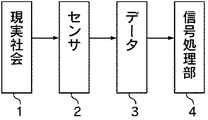•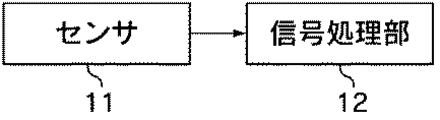•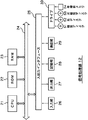•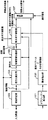•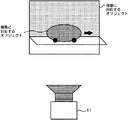•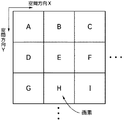••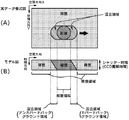••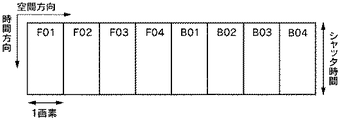•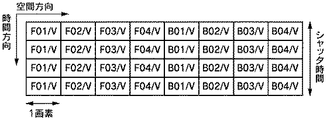•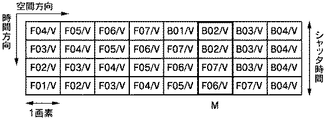•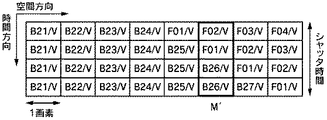•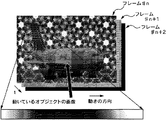•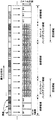•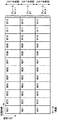•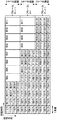•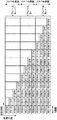•••••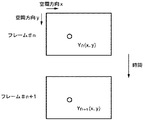•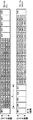•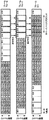•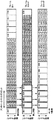•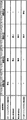•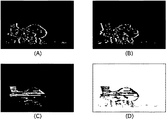••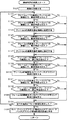•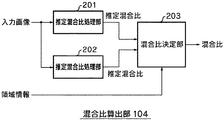•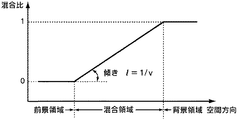•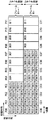•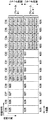•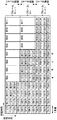•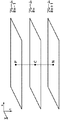••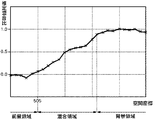••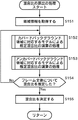••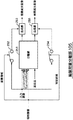••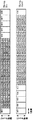•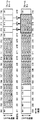•••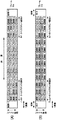•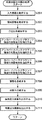•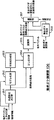••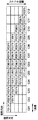•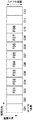•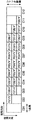•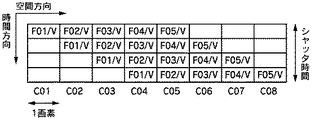•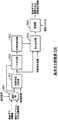•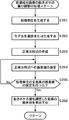•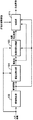•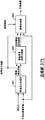•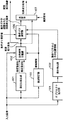•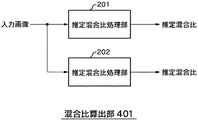•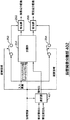•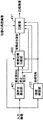•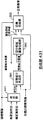## Description


BACKGROUND OF THE INVENTION
The present invention relates to an image processing apparatus and method, and a recording medium, and more particularly, to an image processing apparatus and method that considers a difference between a signal detected by a sensor and the real world, and a recording medium.

[Prior art]
A technique for detecting an event in the real world with a sensor and processing sampling data output from the sensor, such as data corresponding to an image, sound, temperature, pressure, acceleration, or odor, is widely used.

For example, in an image obtained by capturing an object moving in front of a predetermined background with a video camera, motion blur occurs when the moving speed of the object is relatively fast.

Conventionally, in order to suppress such motion blur, for example, the speed of the electronic shutter is increased and the exposure time is shortened.

[Problems to be solved by the invention]
However, this method of increasing the shutter speed requires adjusting the shutter speed of the video camera before imaging. Therefore, there has been a problem that it is impossible to correct a blurred image already obtained and obtain a clear image.

SUMMARY An advantage of some aspects of the invention is that it is possible to adjust the amount of motion blur included in a detection signal such as a blurred image.

[Means for Solving the Problems]
The image processing apparatus according to claim 1 includes: a foreground area including only a foreground object component constituting a foreground object in image data; a background area including only a background object component constituting a background object in image data; A foreground object component and a background object component are mixed in a covered background area formed on the front end side of the foreground object in the moving direction and an uncovered back formed on the rear end side of the foreground object in the moving direction A foreground object from the outer edge of the covered background area centered on the foreground area to the outer edge of the uncovered background area based on the area information indicating the mixed area including the ground area and the image data. A little that matches the direction of movement A processing unit determining means for determining a processing unit composed of pixel data on one straight line, a pixel value of a pixel in the processing unit determined based on the processing unit, and a foreground object component in the mixed region Foreground object component with adjusted motion blur by solving normal equation by means of least squares method and normal equation generating means to generate normal equation by setting division value that is unknown divided by number And a calculation means for generating.

The computing means can generate the foreground object component with the motion blur amount adjusted based on the motion amount of the foreground object.

The computing means can generate the foreground object component from which motion blur is removed based on the amount of motion of the foreground object.

The computing means can adjust the amount of motion blur based on a preset value.

The calculation means can generate a foreground object component with an adjusted motion blur amount by solving a normal equation to calculate a divided value and performing a predetermined calculation process on the divided value.

The image processing apparatus identifies a foreground area, a background area, and a mixed area including a covered background area and an uncovered background area, and includes a foreground area, a background area, and a covered background area and an uncovered background area. A region information generating unit that generates region information indicating the region can be further provided.

The image processing apparatus may further include a mixture ratio detection unit that detects a mixture ratio of at least the foreground object component and the background object component in the mixed region.

The image processing apparatus can further include a separating unit that separates the foreground object and the background object based on the region information and the mixture ratio.

The image processing method according to claim 9 includes: a foreground area including only a foreground object component constituting a foreground object in image data; a background area including only a background object component constituting a background object in image data; A foreground object component and a background object component are mixed in a covered background area formed on the front end side of the foreground object in the moving direction and an uncovered back formed on the rear end side of the foreground object in the moving direction A foreground object from the outer edge of the covered background area centered on the foreground area to the outer edge of the uncovered background area based on the area information indicating the mixed area including the ground area and the image data. A little that matches the direction of movement A processing unit determining step for determining a processing unit composed of pixel data on one straight line, a pixel value of a pixel in the processing unit determined based on the processing unit, and a foreground object component in the mixed region Foreground object component with adjusted amount of motion blur by setting a normal equation generation step that generates a normal equation by setting a division value that is an unknown number divided by a number, and solving the normal equation by the method of least squares And a calculation step of generating

The program of the recording medium according to claim 10 includes: a foreground area including only a foreground object component constituting a foreground object in image data; a background area including only a background object component constituting a background object in image data; and image data. A foreground object component and a background object component are mixed in the foreground object in the moving direction leading end side and the foreground object moving direction rear end side uncovered Based on the area information indicating the mixed area including the background area and the image data, the foreground from the outer edge of the covered background area to the outer edge of the uncovered background area centered on the foreground area. Same as object movement direction A processing unit determining step for determining a processing unit composed of pixel data on at least one straight line, a pixel value of a pixel in the processing unit determined based on the processing unit, and a foreground object component in the mixed area are set. A foreground object in which the amount of motion blur is adjusted by setting a normal equation generation step that generates a normal equation by setting a division value that is an unknown number divided by the number of divisions, and by solving the normal equation by the method of least squares And a calculation step of generating a component.

In the image processing device according to claim 1, the image processing method according to claim 9, and the recording medium according to claim 10, a foreground region including only a foreground object component constituting a foreground object in image data; A background area consisting only of the background object components constituting the background object in the image data, and an area where the foreground object component and the background object component are mixed in the image data, and formed on the front end side in the movement direction of the foreground object. Covered back centered on the foreground area based on the area information indicating the mixed area including the covered background area and the uncovered background area formed on the rear end side in the movement direction of the foreground object and the image data Uncovered back from outside edge of ground area The pixel value of the pixel in the processing unit determined based on the processing unit is determined based on the processing unit determined from the pixel data on the at least one straight line that matches the moving direction of the foreground object up to the outer edge of the round area And the foreground object component in the mixed region are divided by the set number of divisions, and a normal equation is generated, and the normal equation is solved by the method of least squares. Adjusted foreground object components are generated.

DETAILED DESCRIPTION OF THE INVENTION
FIG. 1 illustrates the principle of the present invention. As shown in the figure, a first signal that is information of the real society 1 having a space and a time axis is acquired by the sensor 2 and converted into data. The detection signal which is the data 3 acquired by the sensor 2 is information obtained by projecting information of the real society 1 onto a space-time of a lower dimension than the real society. Therefore, the information obtained by the projection has a distortion generated by the projection. In other words, the data 3 output from the sensor 2 is distorted with respect to information in the real society 1. Data 3 has distortion due to projection, but includes significant information for correcting this.

Therefore, in the present invention, the signal output from the sensor 2 is subjected to signal processing in the signal processing unit 4 so that the distortion is removed, reduced, or adjusted. Alternatively, in the present invention, significant information is extracted by performing signal processing on the data output from the sensor 2 in the signal processing unit 4.

FIG. 2 shows a configuration example of a signal processing apparatus to which the present invention is applied. The sensor 11 is composed of, for example, a video camera, captures a real-world image, and outputs the obtained image data to the signal processing unit 12. The signal processing unit 12 is composed of, for example, a personal computer, processes the data input from the sensor 11, adjusts the amount of distortion generated by the projection, and specifies an area including significant information buried by the projection Or extracting significant information from the specified area, or processing input data based on the extracted significant information.

Significant information here is, for example, a mixture ratio described later.

Note that information indicating an area including significant information buried by projection can also be considered significant information. Here, region information described later corresponds to significant information.

The area including significant information here is, for example, a mixed area described later.

The signal processing unit 12 is configured as shown in FIG. 3, for example. A CPU (Central Processing Uuit) 21 executes various processes according to a program stored in a ROM (Read Only Memory) 22 or a storage unit 28. A RAM (Random Access Memory) 23 appropriately stores programs executed by the CPU 21 and data. The CPU 21, ROM 22, and RAM 23 are connected to each other by a bus 24.

An input / output interface 25 is also connected to the CPU 21 via the bus 24. The input / output interface 25 is connected to an input unit 26 including a keyboard, a mouse, and a microphone, and an output unit 27 including a display and a speaker. The CPU 21 executes various processes in response to commands input from the input unit 26. Then, the CPU 21 outputs an image, sound, or the like obtained as a result of the processing to the output unit 27.

The storage unit 28 connected to the input / output interface 25 is configured by, for example, a hard disk and stores programs executed by the CPU 21 and various data. The communication unit 29 communicates with an external device via the Internet or other networks. In this example, the communication unit 29 functions as an acquisition unit that captures the output of the sensor 11.

A program may be acquired via the communication unit 29 and stored in the storage unit 28.

The drive 30 connected to the input / output interface 25, when a magnetic disk 51, an optical disk 52, a magneto-optical disk 53, or a semiconductor memory 54 is mounted, drives them, and programs and data recorded there. Get etc. The acquired program and data are transferred to and stored in the storage unit 28 as necessary.

Next, a signal processing apparatus that performs processing for identifying a region where significant information is buried or extracting the buried significant information from data acquired by a sensor will be described with a more specific example. In the following example, the CCD line sensor or CCD area sensor corresponds to the sensor, the area information and mixing ratio correspond to significant information, and the foreground and background are mixed and motion blur corresponds to distortion in the mixed area. To do.

FIG. 4 is a block diagram showing the signal processing unit 12.

It does not matter whether each function of the signal processing unit 12 is realized by hardware or software. That is, each block diagram in this specification may be considered as a hardware block diagram or a software functional block diagram.

Here, the motion blur refers to a distortion included in an image corresponding to a moving object, which is caused by the movement of an object in the real world to be imaged and the imaging characteristics of the sensor 11.

In this specification, an image corresponding to an object in the real world to be imaged is referred to as an image object.

The input image supplied to the signal processing unit 12 is supplied to the object extracting unit 101, the region specifying unit 103, the mixture ratio calculating unit 104, and the foreground / background separating unit 105.

The object extraction unit 101 roughly extracts an image object corresponding to a foreground object included in the input image, and supplies the extracted image object to the motion detection unit 102. For example, the object extraction unit 101 detects the outline of the image object corresponding to the foreground object included in the input image, thereby roughly extracting the image object corresponding to the foreground object.

The object extraction unit 101 roughly extracts an image object corresponding to a background object included in the input image, and supplies the extracted image object to the motion detection unit 102. For example, the object extraction unit 101 roughly extracts an image object corresponding to the background object from the difference between the input image and the image object corresponding to the extracted foreground object.

Further, for example, the object extraction unit 101 corresponds to the image object corresponding to the foreground object and the background object from the difference between the background image stored in the background memory provided therein and the input image. You may make it extract the image object to perform roughly.

The motion detection unit 102 calculates the motion vector of the image object corresponding to the coarsely extracted foreground object by a method such as a block matching method, a gradient method, a phase correlation method, and a per-recursive method. The motion vector and the position information of the motion vector (information specifying the position of the pixel corresponding to the motion vector) are supplied to the motion blur extraction unit 106.

The motion vector output from the motion detection unit 102 includes information corresponding to the motion amount v.

Further, for example, the motion detection unit 102 may output a motion vector for each image object to the motion blur adjustment unit 106 together with pixel position information for specifying a pixel in the image object.

The motion amount v is a value that represents a change in the position of the image corresponding to the moving object in units of pixel intervals. For example, when the image of the object corresponding to the foreground is moved so as to be displayed at a position separated by four pixels in the next frame with reference to a certain frame, the motion amount v of the image of the object corresponding to the foreground is 4.

The object extraction unit 101 and the motion detection unit 102 are used when the motion blur adjustment unit 106 performs adjustment of the motion blur amount corresponding to the moving object.

The area specifying unit 103 specifies each pixel of the input image as one of the foreground area, the background area, or the mixed area, and whether each pixel belongs to one of the foreground area, the background area, or the mixed area (Hereinafter referred to as region information) is supplied to the mixture ratio calculation unit 104, foreground / background separation unit 105, and motion blur adjustment unit 106.

Based on the input image and the region information supplied from the region specifying unit 103, the mixture ratio calculation unit 104 calculates a mixture ratio (hereinafter referred to as a mixture ratio α) corresponding to the pixels included in the mixture region 63. The calculated mixture ratio is supplied to the foreground / background separator 105.

The mixing ratio α is a value indicating a ratio of an image component (hereinafter also referred to as a background component) corresponding to a background object in a pixel value, as shown in an equation (3) described later.

Based on the region information supplied from the region specifying unit 103 and the mixture ratio α supplied from the mixture ratio calculation unit 104, the foreground / background separation unit 105 performs image component corresponding to the foreground object (hereinafter referred to as foreground component). The input image is separated into a foreground component image consisting of only the background component and a background component image consisting only of the background component, and the foreground component image is supplied to the motion blur adjustment unit 106 and the selection unit 107. Note that the separated foreground component image may be the final output. Only the foreground and the background can be specified without considering the conventional mixed region, and an accurate foreground and background can be obtained as compared with the separated method.

The motion blur adjustment unit 106 determines a processing unit indicating one or more pixels included in the foreground component image based on the motion amount v and the region information that can be known from the motion vector. The processing unit is data that designates a group of pixels to be subjected to a process for adjusting the amount of motion blur.

The motion blur adjustment unit 106 includes the motion blur adjustment amount input to the signal processing unit 12, the foreground component image supplied from the foreground / background separation unit 105, the motion vector and position information supplied from the motion detection unit 102, and processing. Based on the unit, adjust the amount of motion blur included in the foreground component image, such as removing motion blur included in the foreground component image, reducing the amount of motion blur, or increasing the amount of motion blur. The foreground component image in which the amount of blur is adjusted is output to the selection unit 107. The motion vector and its position information may not be used.

For example, the selection unit 107 adjusts the foreground component image supplied from the foreground / background separation unit 105 and the amount of motion blur supplied from the motion blur adjustment unit 106 based on a selection signal corresponding to the user's selection. One of the foreground component images is selected, and the selected foreground component image is output.

Next, an input image supplied to the signal processing unit 12 will be described with reference to FIGS.

FIG. 5 is a diagram for explaining imaging by a sensor. The sensor 11 is composed of, for example, a CCD video camera provided with a CCD (Charge-Coupled Device) area sensor which is a solid-state imaging device. The object corresponding to the foreground in the real world moves horizontally between the object corresponding to the background and the sensor 11 in the real world, for example, from the left side to the right side in the drawing.

The sensor 11 images an object corresponding to the foreground together with an object corresponding to the background. The sensor 11 outputs the captured image in units of one frame. For example, the sensor 11 outputs an image composed of 30 frames per second. The exposure time of the sensor 11 can be 1/30 second. The exposure time is a period from when the sensor 11 starts converting the input light to electric charge until the conversion of the input light to electric charge ends. Hereinafter, the exposure time is also referred to as shutter time.

FIG. 6 is a diagram illustrating the arrangement of pixels. In FIG. 6, A to I indicate individual pixels. The pixels are arranged on a plane corresponding to the image. One detection element corresponding to one pixel is arranged on the sensor 11. When the sensor 11 captures an image, one detection element outputs a pixel value corresponding to one pixel constituting the image. For example, the position of the detection element in the X direction corresponds to the horizontal position on the image, and the position of the detection element in the Y direction corresponds to the vertical position on the image.

As shown in FIG. 7, for example, a detection element that is a CCD converts input light into electric charges for a period corresponding to a shutter time, and accumulates the converted electric charges. The amount of charge is approximately proportional to the intensity of input light and the time during which light is input. The detection element adds the electric charge converted from the input light to the already accumulated electric charge in a period corresponding to the shutter time. That is, the detection element integrates the input light for a period corresponding to the shutter time, and accumulates an amount of charge corresponding to the integrated light. It can be said that the detection element has an integration effect with respect to time.

The electric charge accumulated in the detection element is converted into a voltage value by a circuit (not shown), and the voltage value is further converted into a pixel value such as digital data and output. Accordingly, each pixel value output from the sensor 11 is a value projected onto a one-dimensional space, which is a result of integrating a part having a spatial extension of the object corresponding to the foreground or the background with respect to the shutter time. Have

The signal processing unit 12 extracts significant information buried in the output signal, for example, the mixing ratio α, by the accumulation operation of the sensor 11. The signal processing unit 12 adjusts the amount of distortion caused by the mixture of the foreground image objects themselves, for example, the amount of motion blur. In addition, the signal processing unit 12 adjusts the amount of distortion caused by the mixture of the foreground image object and the background image object.

FIG. 8 is a diagram for explaining an image obtained by imaging an object corresponding to a moving foreground and an object corresponding to a stationary background. FIG. 8A shows an image obtained by imaging an object corresponding to the foreground with movement and an object corresponding to the stationary background. In the example shown in FIG. 8A, the object corresponding to the foreground is moving horizontally from the left to the right with respect to the screen.

FIG. 8B is a model diagram in which pixel values corresponding to one line of the image shown in FIG. 8A are expanded in the time direction. The horizontal direction in FIG. 8B corresponds to the spatial direction X in FIG.

The pixel value of the background region pixel is composed of only the background component, that is, the image component corresponding to the background object. The pixel value of the foreground region pixel is composed of only the foreground component, that is, the image component corresponding to the foreground object.

The pixel value of the pixel in the mixed area is composed of a background component and a foreground component. Since the pixel value is composed of the background component and the foreground component, the mixed region can be said to be a distortion region. The mixed area is further classified into a covered background area and an uncovered background area.

The covered background area is a mixed area at a position corresponding to the front end of the foreground object in the advancing direction with respect to the foreground area, and is an area where the background component is covered with the foreground as time passes.

On the other hand, the uncovered background area is a mixed area at a position corresponding to the rear end portion of the foreground object in the advancing direction with respect to the foreground area, and an area where a background component appears as time passes. Say.

In this way, an image including a foreground area, a background area, or a covered background area or an uncovered background area is input as an input image to the area specifying unit 103, the mixture ratio calculation unit 104, and the foreground / background separation unit 105. .

FIG. 9 is a diagram illustrating the background area, the foreground area, the mixed area, the covered background area, and the uncovered background area as described above. In the case of corresponding to the image shown in FIG. 8, the background area is a static part, the foreground area is a moving part, the covered background area of the mixed area is a part that changes from the background to the foreground, The uncovered background area is a portion that changes from the foreground to the background.

FIG. 10 is a model diagram in which pixel values of pixels arranged in a row adjacent to each other in an image obtained by capturing an object corresponding to a stationary foreground and an object corresponding to a stationary background are developed in the time direction. It is. For example, pixels arranged on one line of the screen can be selected as the pixels arranged adjacent to each other in one column.

The pixel values F01 to F04 shown in FIG. 10 are pixel values corresponding to the still foreground object. The pixel values B01 to B04 shown in FIG. 10 are pixel values corresponding to the stationary background object.

In the vertical direction in FIG. 10, time elapses from the top to the bottom in the figure. The position of the upper side of the rectangle in FIG. 10 corresponds to the time when the sensor 11 starts to convert the input light into charges, and the position of the lower side of the rectangle in FIG. 10 indicates the position of the light input by the sensor 11. Corresponds to the time when the conversion to charge ends. That is, the distance from the upper side to the lower side of the rectangle in FIG. 10 corresponds to the shutter time.

Hereinafter, a case where the shutter time and the frame interval are the same will be described as an example.

The horizontal direction in FIG. 10 corresponds to the spatial direction X described in FIG. More specifically, in the example shown in FIG. 10, the distance from the left side of the rectangle described as “F01” in FIG. 10 to the right side of the rectangle described as “B04” is 8 times the pixel pitch, That is, it corresponds to the interval between eight consecutive pixels.

When the foreground object and the background object are stationary, the light input to the sensor 11 does not change during the period corresponding to the shutter time.

Here, the period corresponding to the shutter time is divided into two or more periods having the same length. For example, if the number of virtual divisions is 4, the model diagram shown in FIG. 10 can be represented as the model shown in FIG. The virtual division number is set in accordance with the amount of motion v of the object corresponding to the foreground. For example, the number of virtual divisions is 4 corresponding to the motion amount v being 4, and the period corresponding to the shutter time is divided into 4.

The top row in the figure corresponds to the first divided period after the shutter opens. The second row from the top in the figure corresponds to the second divided period from when the shutter has opened. The third line from the top in the figure corresponds to the third divided period from when the shutter has opened. The fourth row from the top in the figure corresponds to the fourth divided period from when the shutter has opened.

Hereinafter, the shutter time divided in accordance with the motion amount v is also referred to as shutter time / v.

Since the light input to the sensor 11 does not change when the object corresponding to the foreground is stationary, the foreground component F01 / v is equal to a value obtained by dividing the pixel value F01 by the virtual division number. Similarly, when the object corresponding to the foreground is stationary, the foreground component F02 / v is equal to the value obtained by dividing the pixel value F02 by the number of virtual divisions, and the foreground component F03 / v is the virtual value of the pixel value F03. The foreground component F04 / v is equal to the value obtained by dividing the pixel value F04 by the virtual division number.

Since the light input to the sensor 11 does not change when the object corresponding to the background is stationary, the background component B01 / v is equal to the value obtained by dividing the pixel value B01 by the virtual division number. Similarly, when the object corresponding to the background is stationary, the background component B02 / v is equal to the value obtained by dividing the pixel value B02 by the virtual division number, and B03 / v is obtained by dividing the pixel value B03 by the virtual division number. B04 / v is equal to a value obtained by dividing the pixel value B04 by the number of virtual divisions.

That is, when the object corresponding to the foreground is stationary, the light corresponding to the foreground object input to the sensor 11 does not change during the period corresponding to the shutter time, so the first shutter time / Corresponds to the foreground component F01 / v corresponding to v, the second foreground component F01 / v corresponding to the shutter time / v, and the third shutter time / v corresponding to the shutter time / v. The foreground component F01 / v and the foreground component F01 / v corresponding to the fourth shutter time / v after the shutter is opened have the same value. F02 / v to F04 / v have the same relationship as F01 / v.

When the object corresponding to the background is stationary, the light corresponding to the background object input to the sensor 11 does not change during the period corresponding to the shutter time, so that the first shutter time / v after the shutter opens. The corresponding background component B01 / v, the second background component B01 / v corresponding to the shutter time / v when the shutter is opened, and the third background component corresponding to the shutter time / v corresponding to the shutter time / v The component B01 / v and the background component B01 / v corresponding to the fourth shutter time / v after the shutter is opened have the same value. B02 / v to B04 / v have the same relationship.

Next, a case where the object corresponding to the foreground moves and the object corresponding to the background is stationary will be described.

FIG. 12 is a model diagram in which pixel values of pixels on one line including the covered background area are expanded in the time direction when the object corresponding to the foreground moves toward the right side in the drawing. In FIG. 12, the foreground motion amount v is 4. Since one frame is a short time, it can be assumed that the object corresponding to the foreground is a rigid body and is moving at a constant speed. In FIG. 12, the image of the object corresponding to the foreground moves so as to be displayed on the right by four pixels in the next frame with reference to a certain frame.

In FIG. 12, the leftmost pixel through the fourth pixel from the left belong to the foreground area. In FIG. 12, the fifth pixel from the left to the seventh pixel from the left belong to the mixed area which is a covered background area. In FIG. 12, the rightmost pixel belongs to the background area.

Since the object corresponding to the foreground is moving so as to cover the object corresponding to the background with the passage of time, the component included in the pixel value of the pixel belonging to the covered background area has a period corresponding to the shutter time. At this point, the background component is replaced by the foreground component.

For example, a pixel value M with a thick frame in FIG. 12 is expressed by Expression (1).

M = B02 / v + B02 / v + F07 / v + F06 / v (1)

For example, since the fifth pixel from the left includes a background component corresponding to one shutter time / v and includes a foreground component corresponding to three shutter times / v, the mixture ratio of the fifth pixel from the left α is 1/4. The sixth pixel from the left includes a background component corresponding to two shutter times / v and includes a foreground component corresponding to two shutter times / v. Therefore, the mixture ratio α of the sixth pixel from the left is 1/2. The seventh pixel from the left includes a background component corresponding to three shutter times / v, and includes a foreground component corresponding to one shutter time / v. Therefore, the mixture ratio α of the seventh pixel from the left is 3/4.

Since it can be assumed that the object corresponding to the foreground is a rigid body and moves at a constant speed so that the foreground image is displayed on the right side of four pixels in the next frame, for example, the fourth pixel from the left in FIG. The foreground component F07 / v of the first shutter time / v after the shutter is opened is the foreground component corresponding to the second shutter time / v of the fifth pixel from the left in FIG. be equivalent to. Similarly, the foreground component F07 / v corresponds to the foreground component of the sixth pixel from the left in FIG. 12 corresponding to the third shutter time / v from when the shutter has opened, and the seventh pixel from the left in FIG. And the foreground component corresponding to the fourth shutter time / v after the shutter is opened.

Since it can be assumed that the object corresponding to the foreground is a rigid body and moves at a constant speed so that the foreground image is displayed on the right side of four pixels in the next frame, for example, the third pixel from the left in FIG. The foreground component F06 / v of the first shutter time / v after the shutter is opened is the foreground component corresponding to the second shutter time / v of the fourth pixel from the left in FIG. equal. Similarly, the foreground component F06 / v is the fifth pixel from the left in FIG. 12 and the foreground component corresponding to the third shutter time / v from when the shutter has opened, and the sixth pixel from the left in FIG. And the foreground component corresponding to the fourth shutter time / v after the shutter is opened.

Since it can be assumed that the object corresponding to the foreground is a rigid body and moves at a constant speed so that the foreground image is displayed on the right side of four pixels in the next frame, for example, the second pixel from the left in FIG. The foreground component F05 / v of the first shutter time / v after the shutter is opened is the foreground component corresponding to the second shutter time / v of the third pixel from the left in FIG. be equivalent to. Similarly, the foreground component F05 / v is the fourth pixel from the left in FIG. 12, the foreground component corresponding to the third shutter time / v from when the shutter is opened, and the fifth pixel from the left in FIG. And the foreground component corresponding to the fourth shutter time / v after the shutter is opened.

Since it can be assumed that the object corresponding to the foreground is a rigid body and moves at a constant speed so that the foreground image is displayed on the right side of four pixels in the next frame, for example, the shutter of the leftmost pixel in FIG. The foreground component F04 / v of the first shutter time / v after opening is equal to the foreground component of the second pixel from the left in FIG. 12 corresponding to the second shutter time / v after the shutter is opened. Similarly, the foreground component F04 / v is the fourth pixel from the left in FIG. 12 and the foreground component of the third pixel from the left in FIG. 12 corresponding to the third shutter time / v from when the shutter has opened. And the foreground component corresponding to the fourth shutter time / v after the shutter is opened.

Since the foreground area corresponding to the moving object includes motion blur as described above, it can be said to be a distortion area.

FIG. 13 is a model diagram in which pixel values of pixels on one line including the uncovered background area are expanded in the time direction when the foreground moves toward the right side in the drawing. In FIG. 13, the foreground motion amount v is 4. Since one frame is a short time, it can be assumed that the object corresponding to the foreground is a rigid body and is moving at a constant speed. In FIG. 13, the image of the object corresponding to the foreground moves to the right by four pixels in the next frame with reference to a certain frame.

In FIG. 13, the leftmost pixel through the fourth pixel from the left belong to the background area. In FIG. 13, the fifth through seventh pixels from the left belong to the mixed area that is an uncovered background. In FIG. 13, the rightmost pixel belongs to the foreground area.

Since the object corresponding to the foreground that covered the object corresponding to the background is moved so as to be removed from the front of the object corresponding to the background over time, it is included in the pixel value of the pixel belonging to the uncovered background area The component to be changed from the foreground component to the background component at a certain point in time corresponding to the shutter time.

For example, the pixel value M ′ with a thick frame in FIG. 13 is expressed by Expression (2).

M '= F02 / v + F01 / v + B26 / v + B26 / v (2)

For example, since the fifth pixel from the left includes a background component corresponding to three shutter times / v and includes a foreground component corresponding to one shutter time / v, the mixture ratio of the fifth pixel from the left α is 3/4. The sixth pixel from the left includes a background component corresponding to two shutter times / v and includes a foreground component corresponding to two shutter times / v. Therefore, the mixture ratio α of the sixth pixel from the left is 1/2. Since the seventh pixel from the left includes a background component corresponding to one shutter time / v and includes a foreground component corresponding to three shutter times / v, the mixture ratio α of the seventh pixel from the left is 1/4.

When the expressions (1) and (2) are generalized, the pixel value M is expressed by the expression (3).

[Expression 1]
Here, α is a mixing ratio. B is a background pixel value, and Fi / v is a foreground component.

Since the object corresponding to the foreground is a rigid body and can be assumed to move at a constant speed and the amount of movement v is 4, for example, the first pixel from the left in FIG. The foreground component F01 / v of the shutter time / v is equal to the foreground component of the sixth pixel from the left in FIG. 13 corresponding to the second shutter time / v after the shutter is opened. Similarly, F01 / v is the foreground component of the seventh pixel from the left in FIG. 13 corresponding to the third shutter time / v after the shutter is opened, and the eighth pixel from the left in FIG. , And the foreground component corresponding to the fourth shutter time / v after the shutter is opened.

Since it can be assumed that the object corresponding to the foreground is a rigid body and moves at a constant speed, and the number of virtual divisions is 4, for example, the first pixel from the left in FIG. The foreground component F02 / v of the shutter time / v is equal to the foreground component of the seventh pixel from the left in FIG. 13 corresponding to the second shutter time / v after the shutter is opened. Similarly, the foreground component F02 / v is equal to the foreground component of the eighth pixel from the left in FIG. 13 corresponding to the third shutter time / v from when the shutter has opened.

Since the object corresponding to the foreground is a rigid body and can be assumed to move at a constant speed and the amount of movement v is 4, for example, the seventh pixel from the left in FIG. The foreground component F03 / v of the shutter time / v is equal to the foreground component of the eighth pixel from the left in FIG. 13 corresponding to the second shutter time / v after the shutter is opened.

In the description of FIG. 11 to FIG. 13, it is described that the virtual division number is 4, but the virtual division number corresponds to the motion amount v. The amount of movement v generally corresponds to the moving speed of the object corresponding to the foreground. For example, when the object corresponding to the foreground is moving so as to be displayed to the right by four pixels in the next frame with reference to a certain frame, the amount of movement v is 4. Corresponding to the motion amount v, the number of virtual divisions is 4. Similarly, for example, when the object corresponding to the foreground is moving so that it is displayed on the left by 6 pixels in the next frame with reference to a certain frame, the motion amount v is set to 6, and the number of virtual divisions is , 6.

14 and 15, the foreground area, the background area, the covered background area or the uncovered background area described above, the foreground components and the background components corresponding to the divided shutter times, and The relationship is shown.

FIG. 14 shows an example in which pixels in the foreground area, background area, and mixed area are extracted from an image including a foreground corresponding to an object moving in front of a stationary background. In the example shown in FIG. 14, the object corresponding to the foreground is moving horizontally with respect to the screen.

Frame # n + 1 is the next frame after frame #n, and frame # n + 2 is the next frame after frame # n + 1.

Extract the pixels in the foreground area, background area, and mixed area extracted from any of frame #n to frame # n + 2, set the amount of motion v to 4, and set the pixel values of the extracted pixels in the time direction The developed model is shown in FIG.

Since the object corresponding to the foreground moves, the pixel value in the foreground area is composed of four different foreground components corresponding to the shutter time / v period. For example, the leftmost pixel among the pixels in the foreground area shown in FIG. 15 is composed of F01 / v, F02 / v, F03 / v, and F04 / v. That is, the pixels in the foreground area include motion blur.

Since the object corresponding to the background is stationary, the light corresponding to the background input to the sensor 11 does not change during the period corresponding to the shutter time. In this case, the pixel value in the background area does not include motion blur.

The pixel value of the pixel belonging to the mixed area composed of the covered background area or the uncovered background area is composed of a foreground component and a background component.

Next, when the image corresponding to the object is moving, the pixel values of the pixels at the same position on the frame that are adjacent to each other in a plurality of frames are developed in the time direction. The model will be described. For example, when the image corresponding to the object moves horizontally with respect to the screen, the pixels arranged on one line of the screen can be selected as the pixels arranged in a row adjacent to each other.

FIG. 16 shows pixels arranged in a row adjacent to three frames of an image obtained by capturing an object corresponding to a stationary background, and the pixel values of the pixels at the same position on the frame are represented by time. It is the model figure developed in the direction. Frame #n is the next frame after frame # n-1, and frame # n + 1 is the next frame after frame #n. Other frames are also referred to in the same manner.

The pixel values B01 to B12 shown in FIG. 16 are pixel values corresponding to a stationary background object. Since the object corresponding to the background is stationary, the pixel value of the corresponding pixel does not change in frame # n−1 to frame n + 1. For example, the pixel in frame #n and the pixel in frame # n + 1 corresponding to the position of the pixel having a pixel value of B05 in frame # n−1 each have a pixel value of B05.

FIG. 17 shows pixels arranged in a row adjacent to three frames of an image obtained by imaging an object corresponding to a foreground moving to the right side in the drawing together with an object corresponding to a stationary background, FIG. 5 is a model diagram in which pixel values of pixels at the same position on a frame are developed in the time direction. The model shown in FIG. 17 includes a covered background area.

In FIG. 17, it can be assumed that the object corresponding to the foreground is a rigid body and moves at a constant speed, and the foreground image is moved so that it is displayed on the right side by four pixels in the next frame. 4 and the number of virtual divisions is 4.

For example, the foreground component of the leftmost pixel of frame # n-1 in FIG. 17 for the first shutter time / v after the shutter opens is F12 / v, and the second pixel from the left in FIG. The foreground component of the second shutter time / v after the shutter is opened is also F12 / v. The foreground component of the third pixel from the left in FIG. 17 for the third shutter time / v after the shutter opens, and the fourth shutter time for the fourth pixel from the left in FIG. The foreground component of / v is F12 / v.

The foreground component of the leftmost pixel of frame # n-1 in FIG. 17 for the second shutter time / v after the shutter opens is F11 / v, and the second pixel from the left in FIG. The foreground component of the third shutter time / v after the shutter is opened is also F11 / v. The foreground component of the third pixel from the left in FIG. 17 corresponding to the fourth portion of the shutter time / v from when the shutter has opened is F11 / v.

The foreground component of the leftmost pixel of frame # n-1 in FIG. 17 for the third shutter time / v from when the shutter has opened is F10 / v, and the second pixel from the left in FIG. The foreground component of the fourth shutter time / v after the shutter is opened is also F10 / v. The foreground component of the leftmost pixel in frame # n−1 in FIG. 17 corresponding to the fourth shutter time / v from when the shutter has opened is F09 / v.

Since the object corresponding to the background is stationary, the background component of the second pixel from the left in frame # n-1 in FIG. 17 corresponding to the first shutter time / v after the shutter opens is B01 / v Become. The background component of the third pixel from the left of frame # n−1 in FIG. 17 corresponding to the first and second shutter time / v from when the shutter has opened is B02 / v. The background component of the fourth pixel from the left of frame # n−1 in FIG. 17 corresponding to the first through third shutter time / v from when the shutter has opened is B03 / v.

In frame # n−1 in FIG. 17, the leftmost pixel belongs to the foreground area, and the second to fourth pixels from the left belong to the mixed area which is a covered background area.

The fifth through twelfth pixels from the left in frame # n−1 in FIG. 17 belong to the background area, and the pixel values thereof are B04 through B11, respectively.

The first through fifth pixels from the left of frame #n in FIG. 17 belong to the foreground area. The foreground component of the shutter time / v in the foreground area of frame #n is any one of F05 / v to F12 / v.

It can be assumed that the object corresponding to the foreground is a rigid body and moves at a constant speed, and the foreground image moves so as to be displayed on the right side of four pixels in the next frame, so from the left of frame #n in FIG. The foreground component of the fifth pixel at the first shutter time / v after the shutter opens is F12 / v, and the sixth pixel from the left in FIG. 17 opens the shutter at the second shutter time / v. The foreground component is also F12 / v. The foreground component of the seventh pixel from the left in FIG. 17 corresponding to the third shutter time / v from when the shutter has opened, and the fourth shutter time from the shutter opening of the eighth pixel from the left in FIG. The foreground component of / v is F12 / v.

The foreground component of the fifth pixel from the left in frame #n in FIG. 17 corresponding to the second shutter time / v from when the shutter has opened is F11 / v, and the sixth pixel from the left in FIG. The foreground component of the third shutter time / v after the shutter is opened is also F11 / v. The foreground component of the seventh pixel from the left in FIG. 17 corresponding to the fourth portion of the shutter time / v from when the shutter has opened is F11 / v.

The foreground component of the fifth pixel from the left in frame #n in FIG. 17 corresponding to the third shutter time / v from when the shutter has opened is F10 / v, and the sixth pixel from the left in FIG. The foreground component of the fourth shutter time / v after the shutter is opened is also F10 / v. The foreground component of the fifth pixel from the left of frame #n in FIG. 17 corresponding to the fourth portion of the shutter time / v from when the shutter has opened is F09 / v.

Since the object corresponding to the background is stationary, the background component of the sixth pixel from the left in frame #n in FIG. 17 corresponding to the first shutter time / v after the shutter is opened is B05 / v. The background component of the seventh pixel from the left of frame #n in FIG. 17 corresponding to the first and second shutter time / v from when the shutter has opened is B06 / v. The background component of the eighth pixel from the left of frame #n in FIG. 17 corresponding to the first through third shutter time / v from when the shutter has opened is B07 / v.

In frame #n in FIG. 17, the sixth through eighth pixels from the left belong to the mixed area, which is a covered background area.

The ninth through twelfth pixels from the left in frame #n in FIG. 17 belong to the background area, and the pixel values are B08 through B11, respectively.

The first through ninth pixels from the left of frame # n + 1 in FIG. 17 belong to the foreground area. The foreground component of the shutter time / v in the foreground area of frame # n + 1 is any one of F01 / v to F12 / v.

It can be assumed that the object corresponding to the foreground is a rigid body and moves at a constant speed, and the foreground image moves so as to be displayed on the right side by four pixels in the next frame. Therefore, the frame # n + 1 in FIG. The foreground component of the ninth pixel from the left when the shutter opens is the first shutter time / v is F12 / v, and the tenth pixel from the left in FIG. 17 is the second shutter time after the shutter is opened. The foreground component of / v is also F12 / v. The foreground component of the eleventh pixel from the left in FIG. 17 and the third shutter time / v from when the shutter has opened, and the fourth shutter time from the shutter of the twelfth pixel from the left in FIG. The foreground component of / v is F12 / v.

The foreground component of the ninth pixel from the left of frame # n + 1 in FIG. 17 corresponding to the second shutter time / v from when the shutter has opened is F11 / v, which is the tenth from the left in FIG. The foreground component of the third shutter time / v after the shutter opens is also F11 / v. The foreground component of the eleventh pixel from the left in FIG. 17 corresponding to the fourth shutter time / v from when the shutter has opened is F11 / v.

The foreground component of the ninth pixel from the left of frame # n + 1 in FIG. 17 corresponding to the third shutter time / v from when the shutter has opened is F10 / v, which is the tenth pixel from the left in FIG. The foreground component of the fourth shutter time / v after the shutter is opened is also F10 / v. The foreground component of the ninth pixel from the left of frame # n + 1 in FIG. 17 corresponding to the fourth portion of the shutter time / v from when the shutter has opened is F09 / v.

Since the object corresponding to the background is stationary, the background component of the tenth pixel from the left of frame # n + 1 in FIG. Become. The background component of the eleventh pixel from the left of frame # n + 1 in FIG. 17 corresponding to the first and second shutter time / v from when the shutter has opened is B10 / v. The background component of the twelfth pixel from the left of frame # n + 1 in FIG. 17 corresponding to the first through third shutter time / v from when the shutter has opened is B11 / v.

In frame # n + 1 in FIG. 17, the tenth through twelfth pixels from the left correspond to the mixed area, which is a covered background area.

FIG. 18 is a model diagram of an image obtained by extracting foreground components from the pixel values shown in FIG.

FIG. 19 shows pixels arranged in a row adjacent to one another in three frames of an image obtained by capturing a foreground corresponding to an object moving to the right side in the drawing together with a stationary background. It is the model figure which expand | deployed the pixel value of the pixel of the position of time direction. In FIG. 19, an uncovered background area is included.

In FIG. 19, it can be assumed that the object corresponding to the foreground is a rigid body and is moving at a constant speed. Since the object corresponding to the foreground is moved so as to be displayed on the right side by four pixels in the next frame, the motion amount v is 4.

For example, the foreground component of the leftmost pixel of frame # n−1 in FIG. 19 that is the first for the shutter time / v after the shutter opens is F13 / v, and is the second pixel from the left in FIG. The foreground component of the second shutter time / v after the shutter is opened is also F13 / v. The foreground component of the third pixel from the left in FIG. 19 corresponding to the third shutter time / v from when the shutter has opened, and the fourth shutter time from the shutter opening of the fourth pixel from the left in FIG. The foreground component of / v is F13 / v.

The foreground component of the second pixel from the left in frame # n-1 in FIG. 19 corresponding to the first shutter time / v from when the shutter has opened is F14 / v, and the third pixel from the left in FIG. The foreground component of the second shutter time / v after the shutter is opened is also F14 / v. The foreground component of the third pixel from the left in FIG. 19 corresponding to the first shutter time / v from when the shutter has opened is F15 / v.

Since the object corresponding to the background is stationary, the background component of the leftmost pixel of frame # n−1 in FIG. 19 corresponding to the second to fourth shutter time / v from when the shutter has opened is B25. / v. The background component of the second pixel from the left of frame # n−1 in FIG. 19 corresponding to the third and fourth shutter time / v from when the shutter has opened is B26 / v. The background component of the third pixel from the left of frame # n−1 in FIG. 19 corresponding to the fourth portion of the shutter time / v from when the shutter has opened is B27 / v.

In frame # n−1 in FIG. 19, the leftmost pixel through the third pixel belong to the mixed area, which is an uncovered background area.

The fourth through twelfth pixels from the left of frame # n−1 in FIG. 19 belong to the foreground area. The foreground component of the frame is any one of F13 / v to F24 / v.

The leftmost pixel through the fourth pixel from the left in frame #n in FIG. 19 belong to the background area, and the pixel values are B25 through B28, respectively.

It can be assumed that the object corresponding to the foreground is a rigid body and moves at a constant speed, and the foreground image moves so as to be displayed on the right side of four pixels in the next frame, so from the left of frame #n in FIG. The foreground component of the fifth pixel at the first shutter time / v after the shutter opens is F13 / v, and the sixth pixel from the left in FIG. The foreground component is also F13 / v. The foreground component of the seventh pixel from the left in FIG. 19 corresponding to the third shutter time / v from when the shutter has opened, and the fourth shutter time from the shutter opening of the eighth pixel from the left in FIG. The foreground component of / v is F13 / v.

The foreground component of the sixth pixel from the left of frame #n in FIG. 19 corresponding to the first shutter time / v from when the shutter has opened is F14 / v, and the seventh pixel from the left in FIG. The foreground component of the second shutter time / v after opening is also F14 / v. The foreground component of the eighth pixel from the left in FIG. 19 corresponding to the first portion of the shutter time / v from when the shutter has opened is F15 / v.

Since the object corresponding to the background is stationary, the background components of the fifth pixel from the left of frame #n in FIG. 19 corresponding to the second through fourth shutter time / v from when the shutter has opened are B29 / v. The background component of the sixth pixel from the left of frame #n in FIG. 19 corresponding to the third and fourth shutter time / v from when the shutter has opened is B30 / v. The background component of the seventh pixel from the left of frame #n in FIG. 19 corresponding to the fourth portion of the shutter time / v from when the shutter has opened is B31 / v.

In frame #n in FIG. 19, the fifth through seventh pixels from the left belong to the mixed area, which is an uncovered background area.

The eighth through twelfth pixels from the left of frame #n in FIG. 19 belong to the foreground area. The value corresponding to the period of the shutter time / v in the foreground area of frame #n is any one of F13 / v to F20 / v.

The leftmost pixel through the eighth pixel from the left in frame # n + 1 in FIG. 19 belong to the background area, and the pixel values thereof are B25 through B32, respectively.

It can be assumed that the object corresponding to the foreground is a rigid body and moves at a constant speed, and moves so that the foreground image is displayed on the right side by four pixels in the next frame. The foreground component of the ninth pixel from the left when the shutter opens is the first shutter time / v is F13 / v, and the tenth pixel from the left in FIG. 19 is the second shutter time after the shutter is opened. The foreground component of / v is also F13 / v. The foreground component of the eleventh pixel from the left in FIG. 19 corresponding to the third shutter time / v from when the shutter has opened, and the fourth shutter time from the shutter opening of the twelfth pixel from the left in FIG. The foreground component of / v is F13 / v.

The foreground component of the tenth pixel from the left of frame # n + 1 in FIG. 19 corresponding to the first shutter time / v from when the shutter has opened is F14 / v, and the eleventh pixel from the left in FIG. The foreground component of the second shutter time / v after the shutter is opened is also F14 / v. The foreground component of the twelfth pixel from the left in FIG. 19 corresponding to the first shutter time / v from when the shutter has opened is F15 / v.

Since the object corresponding to the background is stationary, the background component of the ninth pixel from the left of frame # n + 1 in FIG. , B33 / v. The background component of the tenth pixel from the left of frame # n + 1 in FIG. 19 corresponding to the third and fourth shutter time / v from when the shutter has opened is B34 / v. The background component of the eleventh pixel from the left of frame # n + 1 in FIG. 19 corresponding to the fourth portion of the shutter time / v from when the shutter has opened is B35 / v.

In frame # n + 1 in FIG. 19, the ninth through eleventh pixels from the left belong to the mixed area, which is an uncovered background area.

The twelfth pixel from the left of frame # n + 1 in FIG. 19 belongs to the foreground area. The foreground component of the shutter time / v in the foreground area of frame # n + 1 is any one of F13 / v to F16 / v.

FIG. 20 is a model diagram of an image obtained by extracting foreground components from the pixel values shown in FIG.

Returning to FIG. 4, the region specifying unit 103 associates a flag indicating that the pixel belongs to the foreground region, the background region, the covered background region, or the uncovered background region for each pixel by using the pixel values of a plurality of frames. Thus, the region information is supplied to the mixture ratio calculation unit 104 and the motion blur adjustment unit 106.

The mixture ratio calculation unit 104 calculates the mixture ratio α for each pixel for the pixels included in the mixed region based on the pixel values of a plurality of frames and the region information, and supplies the calculated mixture ratio α to the foreground / background separation unit 105. Supply.

The foreground / background separation unit 105 extracts a foreground component image including only foreground components based on the pixel values of a plurality of frames, region information, and the mixture ratio α, and supplies the foreground component image to the motion blur adjustment unit 106.

The motion blur adjustment unit 106 converts the foreground component image based on the foreground component image supplied from the foreground / background separation unit 105, the motion vector supplied from the motion detection unit 102, and the region information supplied from the region specifying unit 103. The amount of motion blur included is adjusted, and a foreground component image in which the amount of motion blur is adjusted is output.

With reference to the flowchart of FIG. 21, the process of adjusting the amount of motion blur by the signal processing unit 12 will be described. In step S101, the area specifying unit 103 obtains area information indicating whether each pixel of the input image belongs to the foreground area, the background area, the covered background area, or the uncovered background area based on the input image. Execute processing for specifying the area to be generated. Details of the area specifying process will be described later with reference to the flowchart of FIG. The area specifying unit 103 supplies the generated area information to the mixture ratio calculating unit 104.

In step S101, the area specifying unit 103, based on the input image, foreground area, background area, or mixed area for each pixel of the input image (does not distinguish between a covered background area or an uncovered background area). It may be possible to generate region information indicating which one of the items belongs to. In this case, the foreground / background separation unit 105 and the motion blur adjustment unit 106 determine whether the mixed region is a covered background region or an uncovered background region based on the direction of the motion vector. For example, when the foreground region, the mixed region, and the background region are arranged in order corresponding to the direction of the motion vector, the mixed region is determined as the covered background region, and the background is determined corresponding to the direction of the motion vector. When the region, the mixed region, and the foreground region are arranged in this order, the mixed region is determined as an uncovered background region.

In step S102, the mixture ratio calculation unit 104 calculates the mixture ratio α for each pixel included in the mixed area based on the input image and the area information. Details of the mixing ratio calculation process will be described later with reference to the flowchart of FIG. The mixture ratio calculation unit 104 supplies the calculated mixture ratio α to the foreground / background separation unit 105.

In step S103, the foreground / background separator 105 extracts a foreground component from the input image based on the region information and the mixture ratio α, and supplies the foreground component image to the motion blur adjustment unit 106 as a foreground component image.

In step S104, the motion blur adjustment unit 106 is a continuous pixel lined up in the motion direction based on the motion vector and the region information, and belongs to any one of the uncovered background region, the foreground region, and the covered background region. A processing unit indicating the position of the object on the image is generated, and the amount of motion blur included in the foreground component corresponding to the processing unit is adjusted. Details of the process of adjusting the amount of motion blur will be described later with reference to the flowchart of FIG.

In step S105, the signal processing unit 12 determines whether or not the process has been completed for the entire screen. If it is determined that the process has not been completed for the entire screen, the process proceeds to step S104, and the foreground corresponding to the processing unit is determined. The process of adjusting the amount of motion blur for the component is repeated.

If it is determined in step S106 that the process has been completed for the entire screen, the process ends.

As described above, the signal processing unit 12 can adjust the amount of motion blur included in the foreground by separating the foreground and the background. That is, the signal processing unit 12 can adjust the amount of motion blur included in the sample data that is the pixel value of the foreground pixel.

Hereinafter, the configurations of the area specifying unit 103, the mixture ratio calculating unit 104, the foreground / background separating unit 105, and the motion blur adjusting unit 106 will be described.

FIG. 22 is a block diagram illustrating an example of the configuration of the area specifying unit 103. The frame memory 121 stores the input image in units of frames. When the processing target is the frame #n, the frame memory 121 is the frame # n-2 that is the frame two frames before the frame #n, the frame # n-1 that is the frame one frame before the frame #n, A frame #n, a frame # n + 1 that is a frame subsequent to the frame #n, and a frame # n + 2 that is a frame subsequent to the frame #n are stored.

The static determination unit 122-1 determines the pixel value of the pixel of frame # n + 2 at the same position as the position of the pixel that is the target of region specification of frame #n, and the region specification of frame #n. The pixel value of the pixel of frame # n + 1 at the same position as the position of the target pixel on the image is read from the frame memory 121, and the absolute value of the difference between the read pixel values is calculated. The static motion determination unit 122-1 determines whether or not the absolute value of the difference between the pixel value of the frame # n + 2 and the pixel value of the frame # n + 1 is greater than a preset threshold Th, When it is determined that the absolute value of the difference is greater than the threshold value Th, a static motion determination indicating motion is supplied to the region determination unit 123-1. When it is determined that the absolute value of the difference between the pixel value of the pixel of frame # n + 2 and the pixel value of the pixel of frame # n + 1 is equal to or less than the threshold value Th, the static motion determination unit 122-1 The static motion determination shown is supplied to the region determination unit 123-1.

The static motion determination unit 122-2 is the pixel value of the pixel of frame # n + 1 at the same position on the image of the pixel that is the target of region identification of frame #n, and the target of frame #n. The pixel value of the pixel is read from the frame memory 121, and the absolute value of the difference between the pixel values is calculated. The static motion determination unit 122-2 determines whether or not the absolute value of the difference between the pixel value of the frame # n + 1 and the pixel value of the frame #n is greater than a preset threshold value Th. When it is determined that the absolute value of the difference between the two is greater than the threshold value Th, a static motion determination indicating motion is supplied to the region determination unit 123-1 and the region determination unit 123-2. When it is determined that the absolute value of the difference between the pixel value of the pixel of frame # n + 1 and the pixel value of the pixel of frame #n is equal to or less than the threshold value Th, the static motion determination unit 122-2 indicates stillness. The static motion determination is supplied to the region determination unit 123-1 and the region determination unit 123-2.

The static motion determination unit 122-3 generates a frame #n at the same position as the pixel value of the pixel that is the target for specifying the region of frame #n and the position of the pixel that is the target for specifying the region of frame #n. The pixel value of −1 pixel is read from the frame memory 121, and the absolute value of the difference between the pixel values is calculated. The static motion determination unit 122-3 determines whether or not the absolute value of the difference between the pixel value of the frame #n and the pixel value of the frame # n-1 is greater than a preset threshold value Th. When it is determined that the absolute value of the difference between the two is greater than the threshold value Th, a static motion determination indicating motion is supplied to the region determination unit 123-2 and the region determination unit 123-3. When it is determined that the absolute value of the difference between the pixel value of the pixel of frame #n and the pixel value of the pixel of frame # n-1 is equal to or less than the threshold value Th, the static motion determination unit 122-3 indicates the still state The static motion determination is supplied to the region determination unit 123-2 and the region determination unit 123-3.

The static motion determination unit 122-4 determines the pixel value of the pixel of frame # n-1 at the same position on the image of the pixel that is the target of region specification of frame #n, and the region specification of frame #n. The pixel value of the pixel of frame # n-2 at the same position on the image of the target pixel is read from the frame memory 121, and the absolute value of the difference between the pixel values is calculated. The static motion determination unit 122-4 determines whether the absolute value of the difference between the pixel value of the frame # n-1 and the pixel value of the frame # n-2 is greater than a preset threshold Th, When it is determined that the absolute value of the difference between the pixel values is greater than the threshold value Th, a static motion determination indicating motion is supplied to the region determination unit 123-3. When the absolute value of the difference between the pixel value of the pixel of frame # n-1 and the pixel value of the pixel of frame # n-2 is determined to be equal to or less than the threshold value Th, the static motion determination unit 122-4 Is supplied to the region determination unit 123-3.

The region determination unit 123-1 is configured such that the static motion determination supplied from the static motion determination unit 122-1 indicates stillness and the static motion determination supplied from the static motion determination unit 122-2 indicates movement. The pixel that is the target of region identification in frame #n is determined to belong to the uncovered background region, and the uncovered background region determination flag corresponding to the pixel that is determined to belong to the region belongs to the uncovered background region. “1” indicating “” is set.

In the region determination unit 123-1, the static motion determination supplied from the static motion determination unit 122-1 indicates movement, or the static motion determination supplied from the static motion determination unit 122-2 indicates static. When the pixel that is the target of region identification in frame #n is determined not to belong to the uncovered background region, the uncovered background region determination flag corresponding to the pixel to be determined for the region is set to the uncovered background region. “0” is set to indicate that it does not belong.

The area determination unit 123-1 supplies the uncovered background area determination flag in which “1” or “0” is set in this way to the determination flag storage frame memory 124.

The area determination unit 123-2 is configured such that the static motion determination supplied from the static motion determination unit 122-2 indicates static and the static motion determination supplied from the static motion determination unit 122-3 indicates static. Then, it is determined that the pixel that is the target of region identification in frame #n belongs to the still region, and “1” indicating that it belongs to the still region is set in the still region determination flag corresponding to the pixel to be determined for the region.

In the area determination unit 123-2, the static motion determination supplied from the static motion determination unit 122-2 indicates a motion, or the static motion determination supplied from the static motion determination unit 122-3 indicates a motion. At this time, it is determined that the pixel that is the region identification target in frame #n does not belong to the still region, and “0” indicating that it does not belong to the still region is set in the still region determination flag corresponding to the pixel to be determined for the region. Set.

The region determination unit 123-2 supplies the still region determination flag set to “1” or “0” to the determination flag storage frame memory 124 as described above.

The region determination unit 123-2 is configured such that the static motion determination supplied from the static motion determination unit 122-2 indicates movement and the static motion determination supplied from the static motion determination unit 122-3 indicates movement. Then, it is determined that the pixel that is the target of region identification in frame #n belongs to the motion region, and “1” indicating that it belongs to the motion region is set in the motion region determination flag corresponding to the pixel determined for the region.

In the region determination unit 123-2, the static motion determination supplied from the static motion determination unit 122-2 indicates static or the static motion determination supplied from the static motion determination unit 122-3 indicates static. At this time, it is determined that the pixel that is the region identification target in frame #n does not belong to the motion region, and “0” indicating that it does not belong to the motion region is set in the motion region determination flag corresponding to the pixel that is determined to be the region. Set.

The region determination unit 123-2 supplies the motion region determination flag set to “1” or “0” to the determination flag storage frame memory 124 in this way.

The region determination unit 123-3 is configured such that the static motion determination supplied from the static motion determination unit 122-3 indicates movement and the static motion determination supplied from the static motion determination unit 122-4 indicates stillness. , It is determined that the pixel that is the target of region identification in frame #n belongs to the covered background region, and the covered background region determination flag corresponding to the pixel to be determined of the region indicates that it belongs to the covered background region. 1 ”is set.

In the area determination unit 123-3, the static motion determination supplied from the static motion determination unit 122-3 indicates stillness, or the static motion determination supplied from the static motion determination unit 122-4 indicates movement. When determining that the pixel for which the region is specified in frame #n does not belong to the covered background region, the covered background region determination flag corresponding to the pixel to be determined does not belong to the covered background region. “0” is set to indicate.

The area determination unit 123-3 supplies the covered background area determination flag set to “1” or “0” to the determination flag storage frame memory 124 as described above.

The determination flag storage frame memory 124 is supplied from the uncovered background region determination flag supplied from the region determination unit 123-1, the still region determination flag supplied from the region determination unit 123-2, and the region determination unit 123-2. The motion area determination flag and the covered background area determination flag supplied from the area determination unit 123-3 are stored.

The determination flag storage frame memory 124 supplies the stored uncovered background area determination flag, still area determination flag, motion area determination flag, and covered background area determination flag to the synthesis unit 125. Based on the uncovered background area determination flag, the still area determination flag, the motion area determination flag, and the covered background area determination flag supplied from the determination flag storage frame memory 124, the synthesis unit 125 Area information indicating that it belongs to any one of the covered background area, the stationary area, the motion area, and the covered background area is generated and supplied to the determination flag storage frame memory 126.

The determination flag storage frame memory 126 stores the region information supplied from the combining unit 125 and outputs the stored region information.

Next, an example of processing of the area specifying unit 103 will be described with reference to FIGS.

When the object corresponding to the foreground is moving, the position on the screen of the image corresponding to the object changes for each frame. As shown in FIG. 23, in the frame #n, the image corresponding to the object located at the position indicated by Yn (x, y) is Yn + 1 (x, Located in y).

FIG. 24 shows a model diagram in which pixel values of pixels arranged in a row adjacent to the moving direction of the image corresponding to the foreground object are developed in the time direction. For example, when the moving direction of the image corresponding to the foreground object is horizontal with respect to the screen, the model diagram in FIG. 24 shows a model in which pixel values of adjacent pixels on one line are expanded in the time direction.

In FIG. 24, the line in frame #n is the same as the line in frame # n + 1.

Foreground components corresponding to the objects included in the second through thirteenth pixels from the left in frame #n are included in the sixth through seventeenth pixels from the left in frame # n + 1.

In frame #n, the pixels belonging to the covered background area are the 11th to 13th pixels from the left, and the pixels belonging to the uncovered background area are the 2nd to 4th pixels from the left. In frame # n + 1, the pixels belonging to the covered background area are the 15th to 17th pixels from the left, and the pixels belonging to the uncovered background area are the 6th to 8th pixels from the left.

In the example shown in FIG. 24, the foreground component included in frame #n has moved four pixels in frame # n + 1, so the amount of motion v is four. The virtual division number corresponds to the motion amount v and is 4.

Next, changes in pixel values of pixels belonging to the mixed region before and after the frame of interest will be described.

In the frame #n shown in FIG. 25 where the background is stationary and the foreground motion amount v is 4, the pixels belonging to the covered background area are the fifteenth through seventeenth pixels from the left. Since the motion amount v is 4, in the previous frame # n−1, the fifteenth through seventeenth pixels from the left include only background components and belong to the background area. In frame # n-2, the fifteenth through seventeenth pixels from the left include only background components and belong to the background area.

Here, since the object corresponding to the background is stationary, the pixel value of the fifteenth pixel from the left in frame # n-1 does not change from the pixel value of the fifteenth pixel from the left in frame # n-2. . Similarly, the pixel value of the 16th pixel from the left of frame # n-1 does not change from the pixel value of the 16th pixel from the left of frame # n-2, and the 17th pixel from the left of frame # n-1 The pixel value of this pixel does not change from the pixel value of the 17th pixel from the left in frame # n-2.

That is, the pixels of frame # n-1 and frame # n-2 corresponding to the pixels belonging to the covered background area in frame #n are composed of only background components, and the pixel value does not change. The value is almost zero. Therefore, the static motion determination for the pixels in frame # n-1 and frame # n-2 corresponding to the pixels belonging to the mixed region in frame #n is determined as static by the static motion determination unit 122-4.

Since the pixels belonging to the covered background area in frame #n include the foreground components, the pixel values are different from the case of only the background components in frame # n-1. Therefore, the static motion determination for the pixels belonging to the mixed region in frame #n and the corresponding pixels in frame # n-1 is determined as motion by the static motion determination unit 122-3.

As described above, the region determination unit 123-3 is supplied with the result of the static motion determination indicating the motion from the static motion determination unit 122-3, and is supplied with the result of the static motion determination indicating the static motion from the static motion determination unit 122-4. When it is done, it is determined that the corresponding pixel belongs to the covered background area.

In frame #n shown in FIG. 26 where the background is stationary and the foreground motion amount v is 4, the pixels included in the uncovered background area are the second through fourth pixels from the left. Since the motion amount v is 4, in the next frame # n + 1, the second through fourth pixels from the left include only background components and belong to the background area. Further, in the next frame # n + 2, the second through fourth pixels from the left include only background components and belong to the background area.

Here, since the object corresponding to the background is stationary, the pixel value of the second pixel from the left of frame # n + 2 does not change from the pixel value of the second pixel from the left of frame # n + 1. . Similarly, the pixel value of the third pixel from the left of frame # n + 2 does not change from the pixel value of the third pixel from the left of frame # n + 1, and is the fourth from the left of frame # n + 2. The pixel value of this pixel does not change from the pixel value of the fourth pixel from the left in frame # n + 1.

That is, the pixels of frame # n + 1 and frame # n + 2, which correspond to the pixels belonging to the uncovered background area in frame #n, consist only of background components, and the pixel value does not change. The absolute value is almost zero. Accordingly, the static motion determination for the pixels of frame # n + 1 and frame # n + 2 corresponding to the pixels belonging to the mixed area in frame #n is determined as static by the static motion determination unit 122-1.

Since the pixels belonging to the uncovered background area in frame #n include the foreground components, the pixel values are different from the case of only the background components in frame # n + 1. Therefore, the static motion determination for the pixels belonging to the mixed region in frame #n and the corresponding pixels in frame # n + 1 is determined as motion by the static motion determination unit 122-2.

As described above, the region determination unit 123-1 is supplied with the result of the static motion determination indicating the motion from the static motion determination unit 122-2, and is supplied with the result of the static motion determination indicating the static motion from the static motion determination unit 122-1. Is determined to belong to the uncovered background area.

FIG. 27 is a diagram illustrating determination conditions of the area specifying unit 103 in frame #n. The pixel in frame # n-2 at the same position on the image of the pixel to be judged in frame #n and the same position on the image of the pixel to be judged in frame #n A pixel in frame # n-1 is determined to be stationary, and a pixel in frame # n-1 and a pixel in frame #n at the same position on the image of the pixel to be determined in frame #n Are determined to be movements, the area specifying unit 103 determines that the pixel to be determined for frame #n belongs to the covered background area.

The pixel in frame # n-1 and the pixel in frame #n at the same position on the image of the pixel to be determined in frame #n are determined to be stationary, and the pixel in frame #n When it is determined that the pixel of frame # n + 1 at the same position on the image of the pixel to be determined as #n is still, the area specifying unit 103 determines that the determination target of frame #n is Is determined to belong to the still region.

The pixel in frame # n-1 and the pixel in frame #n at the same position on the image of the pixel to be determined in frame #n are determined to move, and the pixel in frame #n When it is determined that a pixel in frame # n + 1 located at the same position on the image of a pixel to be determined as #n is a motion, the region specifying unit 103 determines that the determination is performed in frame #n. Is determined to belong to the motion region.

The pixel in frame #n and the pixel in frame # n + 1 at the same position on the image of the pixel to be determined in frame #n are determined as motion, and the determination target in frame #n The pixel of frame # n + 1 at the same position as the position of the pixel on the image and the pixel of frame # n + 2 at the same position as the position of the pixel to be determined at frame #n on the image Are determined to be stationary, the area specifying unit 103 determines that the pixel to be determined for frame #n belongs to the uncovered background area.

FIG. 28 is a diagram illustrating an example of the result of specifying the area of the area specifying unit 103. In FIG. 28A, pixels determined to belong to the covered background area are displayed in white. In FIG. 28B, pixels determined to belong to the uncovered background area are displayed in white.

In FIG. 28C, pixels determined to belong to the motion region are displayed in white. In FIG. 28D, the pixels determined to belong to the still area are displayed in white.

FIG. 29 is a diagram showing, as an image, area information indicating a mixed area among the area information output from the determination flag storage frame memory 126. In FIG. 29, a pixel determined to belong to the covered background area or the uncovered background area, that is, a pixel determined to belong to the mixed area is displayed in white. The area information indicating the mixed area output from the determination flag storage frame memory 126 indicates a mixed area and a textured part surrounded by a non-textured part in the foreground area.

Next, the area specifying process of the area specifying unit 103 will be described with reference to the flowchart of FIG. In step S121, the frame memory 121 acquires images of frames # n-2 to # n + 2 including the frame #n to be determined.

In step S122, the static motion determination unit 122-3 determines whether the pixel in frame # n-1 and the pixel at the same position in frame #n are stationary. Then, the static motion determination unit 122-2 determines whether or not the frame #n and the pixel at the same position in the frame # n + 1 are still.

If it is determined in step S123 that the pixel in frame #n and the pixel in the same position in frame # n + 1 are determined to be stationary, the process proceeds to step S124, and the region determination unit 123-2 determines that the region is determined as a pixel. A corresponding still area determination flag is set to “1” indicating that it belongs to a still area. The region determination unit 123-2 supplies the still region determination flag to the determination flag storage frame memory 124, and the procedure proceeds to step S125.

When it is determined in step S122 that the pixel in frame # n-1 and the pixel in the same position in frame #n are in motion, or in step S123, the pixel in frame #n and the same position in frame # n + 1 If the pixel is determined to be moving, the pixel of frame #n does not belong to the still region, so the process of step S124 is skipped, and the procedure proceeds to step S125.

In step S125, the static motion determination unit 122-3 determines whether or not the pixel in frame # n-1 and the pixel in the same position in frame #n are in motion, and if it is determined as motion, the process proceeds to step S126. Proceeding, the static motion determination unit 122-2 determines whether or not there is motion between the pixel of frame #n and the pixel at the same position of frame # n + 1.

If it is determined in step S126 that the motion of the pixel in frame #n and the pixel in the same position in frame # n + 1 is determined as moving, the process proceeds to step S127, and the region determination unit 123-2 determines that the region is determined. “1” indicating that it belongs to a motion region is set in the corresponding motion region determination flag. The region determination unit 123-2 supplies the motion region determination flag to the determination flag storage frame memory 124, and the procedure proceeds to step S128.

If it is determined in step S125 that the pixel in frame # n-1 and the pixel in the same position in frame #n are stationary, or in step S126, the pixel in frame #n and the same position in frame # n + 1 If the current pixel is determined to be still, the pixel of frame #n does not belong to the motion region, so the process of step S127 is skipped, and the procedure proceeds to step S128.

In step S128, the static motion determination unit 122-4 determines whether or not the pixel in frame # n-2 and the pixel at the same position in frame # n-1 are stationary. Proceeding to S129, the static motion determination unit 122-3 determines whether or not there is movement between the pixel of frame # n-1 and the pixel at the same position of frame #n.

If it is determined in step S129 that the pixel in frame # n-1 and the pixel in the same position in frame #n are in motion, the process proceeds to step S130, and the region determination unit 123-3 determines that the region is to be determined. The corresponding covered background area determination flag is set to “1” indicating that it belongs to the covered background area. The region determination unit 123-3 supplies the covered background region determination flag to the determination flag storage frame memory 124, and the procedure proceeds to step S131.

If it is determined in step S128 that the pixel in frame # n-2 and the pixel in the same position in frame # n-1 are in motion, or in step S129, the pixel in frame # n-1 and the pixel in frame #n If it is determined that the pixel at the same position is still, the pixel of frame #n does not belong to the covered background area, so the process of step S130 is skipped, and the procedure proceeds to step S131.

In step S131, the static motion determination unit 122-2 determines whether or not the pixel in the frame #n and the pixel in the same position in the frame # n + 1 are in motion, and if it is determined as motion, the process proceeds to step S132. Then, the static motion determination unit 122-1 determines whether or not the frame # n + 1 and the pixel at the same position in the frame # n + 2 are still.

If it is determined in step S132 that the pixel in frame # n + 1 and the pixel in the same position in frame # n + 2 are stationary, the process proceeds to step S133, and the region determination unit 123-1 determines the region. In the uncovered background area determination flag corresponding to the pixel, “1” indicating that the pixel belongs to the uncovered background area is set. The region determination unit 123-1 supplies the uncovered background region determination flag to the determination flag storage frame memory 124, and the procedure proceeds to step S134.

If it is determined in step S131 that the pixel in frame #n and the pixel at the same position in frame # n + 1 are stationary, or in step S132, the pixel in frame # n + 1 and the frame # n + 2 If it is determined that the motion is the same pixel, the pixel of frame #n does not belong to the uncovered background area, so the process of step S133 is skipped, and the procedure proceeds to step S134.

In step S134, the area specifying unit 103 determines whether or not an area has been specified for all the pixels of frame #n, and if it is determined that no area has been specified for all the pixels of frame #n, Returns to step S122 and repeats the area specifying process for other pixels.

If it is determined in step S134 that the area has been specified for all the pixels of frame #n, the process proceeds to step S135, where the synthesis unit 125 stores the uncovered background area determination flag stored in the determination flag storage frame memory 124. And a covered background area determination flag, area information indicating a mixed area is generated, and each pixel belongs to one of an uncovered background area, a stationary area, a motion area, and a covered background area. The region information indicating this is generated, the generated region information is set in the determination flag storage frame memory 126, and the process ends.

As described above, the region specifying unit 103 may generate region information indicating that each pixel included in the frame belongs to the motion region, the still region, the uncovered background region, or the covered background region. it can.

The area specifying unit 103 generates area information corresponding to the mixed area by applying a logical sum to the area information corresponding to the uncovered background area and the covered background area, and is included in the frame. For each pixel, region information including a flag indicating that the pixel belongs to a motion region, a still region, or a mixed region may be generated.

When the object corresponding to the foreground has a texture, the area specifying unit 103 can specify the movement area more accurately.

The area specifying unit 103 can output area information indicating a motion area as area information indicating a foreground area, and area information indicating a still area as area information indicating a background area.

In addition, although the object corresponding to the background has been described as stationary, the above-described processing for specifying the region can be applied even if the image corresponding to the background region includes a motion. For example, when the image corresponding to the background area is moving uniformly, the area specifying unit 103 shifts the entire image corresponding to this movement, and performs the same processing as when the object corresponding to the background is stationary. To do. In addition, when the image corresponding to the background region includes a different motion for each local area, the region specifying unit 103 selects a pixel corresponding to the motion and executes the above-described processing.

FIG. 31 is a block diagram illustrating an example of the configuration of the mixture ratio calculation unit 104. The estimated mixture ratio processing unit 201 calculates an estimated mixture ratio for each pixel by an operation corresponding to the model of the covered background region based on the input image, and supplies the calculated estimated mixture ratio to the mixture ratio determining unit 203. To do.

The estimated mixture ratio processing unit 202 calculates an estimated mixture ratio for each pixel by an operation corresponding to the model of the uncovered background area based on the input image, and sends the calculated estimated mixture ratio to the mixture ratio determination unit 203. Supply.

Since it can be assumed that the object corresponding to the foreground is moving at a constant speed within the shutter time, the mixture ratio α of the pixels belonging to the mixed area has the following properties. That is, the mixture ratio α changes linearly in response to changes in the pixel position. If the change in the pixel position is one-dimensional, the change in the mixture ratio α can be expressed by a straight line. If the change in the pixel position is two-dimensional, the change in the mixture ratio α is expressed by a plane. be able to.

Since the period of one frame is short, it is assumed that the object corresponding to the foreground is a rigid body and is moving at a constant speed.

In this case, the gradient of the mixture ratio α is the inverse ratio of the motion amount v within the foreground shutter time.

An example of an ideal mixing ratio α is shown in FIG. The gradient l in the mixing region of the ideal mixing ratio α can be expressed as the reciprocal of the motion amount v.

As shown in FIG. 32, the ideal mixture ratio α has a value of 1 in the background region, a value of 0 in the foreground region, and a value greater than 0 and less than 1 in the mixed region. .

In the example of FIG. 33, the pixel value C06 of the seventh pixel from the left of frame #n can be expressed by Expression (4) using the pixel value P06 of the seventh pixel from the left of frame # n-1. it can.

[Expression 2]

In Expression (4), the pixel value C06 is expressed as the pixel value M of the pixel in the mixed region, and the pixel value P06 is expressed as the pixel value B of the pixel in the background region. That is, the pixel value M of the pixel in the mixed region and the pixel value B of the pixel in the background region can be expressed as Equation (5) and Equation (6), respectively.

M = C06 (5)
B = P06 (6)

2 / v in equation (4) corresponds to the mixing ratio α. Since the motion amount v is 4, the mixture ratio α of the seventh pixel from the left of the frame #n is 0.5.

As described above, the pixel value C of the focused frame #n is regarded as the pixel value of the mixed region, and the pixel value P of the frame # n-1 before the frame #n is regarded as the pixel value of the background region. Equation (3) indicating the mixing ratio α can be rewritten as Equation (7).

C = α ・ P + f (7)
F in Expression (7) is the sum of the foreground components included in the pixel of interest Σ i Fi / v. There are two variables included in Equation (7): the mixture ratio α and the sum f of the foreground components.

Similarly, FIG. 34 shows a model in which pixel values are expanded in the time direction, in which the amount of motion v is 4 and the number of virtual divisions in the time direction is 4, in the uncovered background area.

In the uncovered background area, similarly to the above-described expression in the covered background area, the pixel value C of the frame #n of interest is regarded as the pixel value of the mixed area, and the frame # n + 1 after the frame #n Eq. (3) indicating the mixture ratio α can be expressed as Eq. (8) by regarding the pixel value N of と as the pixel value of the background region.

C = α ・ N + f (8)

Although it has been described that the background object is stationary, even when the background object is moving, by using the pixel value of the pixel at the position corresponding to the background motion amount v, the expression (4 ) To formula (8) can be applied. For example, in FIG. 33, when the motion amount v of the object corresponding to the background is 2 and the number of virtual divisions is 2, when the object corresponding to the background is moving to the right side in the figure, The pixel value B of the pixel in the background area is set to a pixel value P04.

Since Expression (7) and Expression (8) each include two variables, the mixture ratio α cannot be obtained as it is. Here, since an image generally has a strong spatial correlation, adjacent pixels have almost the same pixel value.

Therefore, since the foreground components have a strong spatial correlation, the formula is modified so that the sum f of the foreground components can be derived from the previous or subsequent frame to obtain the mixture ratio α.

The pixel value Mc of the seventh pixel from the left in frame #n in FIG. 35 can be expressed by Expression (9).

[Equation 3]
2 / v in the first term on the right side of Equation (9) corresponds to the mixing ratio α. The second term on the right side of Expression (9) is expressed as Expression (10) using the pixel value of the subsequent frame # n + 1.

[Expression 4]

Here, Equation (11) is established using the spatial correlation of the foreground components.

F = F05 = F06 = F07 = F08 = F09 = F10 = F11 = F12 (11)
Expression (10) can be replaced with Expression (12) using Expression (11).

[Equation 5]

As a result, β can be expressed by equation (13).

β = 2/4 (13)

In general, assuming that the foreground components related to the mixed area are equal as shown in Expression (11), Expression (14) is established from the relationship of the internal ratio for all the pixels in the mixed area.

β = 1-α (14)

If Expression (14) is established, Expression (7) can be expanded as shown in Expression (15).

[Formula 6]

Similarly, if equation (14) holds, equation (8) can be expanded as shown in equation (16).

[Expression 7]

In Expressions (15) and (16), C, N, and P are known pixel values, so the only variable included in Expressions (15) and (16) is the mixture ratio α. FIG. 36 shows the relationship between C, N, and P in the equations (15) and (16). C is the pixel value of the pixel of interest in frame #n for calculating the mixture ratio α. N is a pixel value of a pixel in frame # n + 1 corresponding to a pixel of interest corresponding to a position in the spatial direction. P is a pixel value of a pixel in frame # n−1 in which the pixel of interest corresponds to the position in the spatial direction.

Therefore, since one variable is included in each of Expression (15) and Expression (16), the mixture ratio α can be calculated using the pixel values of the pixels of the three frames. The condition for calculating the correct mixture ratio α by solving the equations (15) and (16) is that the foreground components related to the mixed region are equal, that is, when the foreground object is stationary. In the foreground image object thus obtained, the pixel values of the pixels located at the boundary of the image object corresponding to the direction of the motion of the foreground object, which are twice as many as the movement amount v, are continuous. It is constant.

As described above, the mixture ratio α of pixels belonging to the covered background area is calculated by Expression (17), and the mixture ratio α of pixels belonging to the uncovered background area is calculated by Expression (18).

α = (CN) / (PN) (17)
α = (CP) / (NP) (18)

FIG. 37 is a block diagram illustrating a configuration of the estimated mixture ratio processing unit 201. The frame memory 221 stores the input image in units of frames, and supplies the frame immediately after the frame input as the input image to the frame memory 222 and the mixture ratio calculation unit 223.

The frame memory 222 stores the input image in units of frames, and supplies the frame immediately after the frame supplied from the frame memory 221 to the mixing ratio calculation unit 223.

Therefore, when the frame # n + 1 is input to the mixture ratio calculation unit 223 as an input image, the frame memory 221 supplies the frame #n to the mixture ratio calculation unit 223, and the frame memory 222 stores the frame # n− 1 is supplied to the mixture ratio calculation unit 223.

The mixture ratio calculation unit 223 calculates the pixel value C of the pixel of interest in frame #n and the pixel of frame # n + 1 corresponding to the spatial position of the pixel of interest by the calculation shown in Expression (17). And the estimated mixture ratio of the pixel of interest was calculated based on the pixel value N of the pixel and the pixel value P of the pixel of frame # n-1 whose spatial position corresponds to the pixel of interest. Output the estimated mixture ratio. For example, when the background is stationary, the mixture ratio calculation unit 223 determines that the pixel value C of the pixel of interest in frame #n is the same as the pixel of interest in the frame # n + 1, and the position in the frame is the same. Calculate the estimated mixture ratio of the pixel of interest based on the pixel value N of the pixel and the pixel value P of the pixel in frame # n-1, which has the same position in the frame as the pixel of interest. The estimated mixture ratio is output.

As described above, the estimated mixture ratio processing unit 201 can calculate the estimated mixture ratio based on the input image and supply the estimated mixture ratio to the mixture ratio determining unit 203.

The estimated mixture ratio processing unit 202 calculates the estimated mixture ratio of the pixel of interest by the calculation shown in the equation (17) by the estimated mixture ratio processing unit 201, whereas the calculation shown in the equation (18). Therefore, except that the part for calculating the estimated mixture ratio of the pixel of interest is different, it is the same as the estimated mixture ratio processing unit 201, and the description thereof will be omitted.

FIG. 38 is a diagram illustrating an example of the estimated mixture ratio calculated by the estimated mixture ratio processing unit 201. The estimated mixture ratio shown in FIG. 38 indicates the result when the foreground motion amount v corresponding to an object moving at a constant speed is 11, for one line.

It can be seen that the estimated mixture ratio changes almost linearly in the mixed region as shown in FIG.

Referring back to FIG. 31, the mixture ratio determining unit 203 determines that the pixel for which the mixture ratio α supplied from the region specifying unit 103 is to be calculated is a foreground area, a background area, a covered background area, or an uncovered background area. The mixing ratio α is set on the basis of the area information indicating which of the two. The mixture ratio determining unit 203 sets 0 as the mixture ratio α when the target pixel belongs to the foreground area, and sets 1 as the mixture ratio α when the target pixel belongs to the background area. When the pixel belongs to the covered background area, the estimated mixture ratio supplied from the estimated mixture ratio processing unit 201 is set to the mixture ratio α, and when the target pixel belongs to the uncovered background area, the estimated mixture ratio processing unit The estimated mixture ratio supplied from 202 is set to the mixture ratio α. The mixture ratio determination unit 203 outputs a mixture ratio α set based on the region information.

FIG. 39 is a block diagram showing another configuration of the mixture ratio calculation unit 104. Based on the region information supplied from the region specifying unit 103, the selection unit 231 supplies the pixels belonging to the covered background region and the corresponding pixels of the previous and subsequent frames to the estimated mixture ratio processing unit 232. Based on the region information supplied from the region specifying unit 103, the selection unit 231 supplies the pixels belonging to the uncovered background region and the corresponding pixels in the previous and subsequent frames to the estimated mixture ratio processing unit 233. .

The estimated mixture ratio processing unit 232 calculates the estimated mixture ratio of the pixel of interest belonging to the covered background area by the calculation shown in Expression (17) based on the pixel value input from the selection unit 231. The calculated estimated mixture ratio is supplied to the selection unit 234.

The estimated mixture ratio processing unit 233 calculates the estimated mixture ratio of the pixel of interest belonging to the uncovered background area by the calculation shown in Expression (18) based on the pixel value input from the selection unit 231. Then, the calculated estimated mixture ratio is supplied to the selection unit 234.

When the target pixel belongs to the foreground area based on the area information supplied from the area specifying unit 103, the selection unit 234 selects an estimated mixture ratio that is 0, sets the mixture ratio α, If the pixel belongs to the background region, an estimated mixture ratio of 1 is selected and set to the mixture ratio α. When the target pixel belongs to the covered background area, the selection unit 234 selects the estimated mixture ratio supplied from the estimated mixture ratio processing unit 232 and sets it to the mixture ratio α, and the target pixel is uncovered back. When belonging to the ground region, the estimated mixture ratio supplied from the estimated mixture ratio processing unit 233 is selected and set to the mixture ratio α. The selection unit 234 outputs the mixture ratio α selected and set based on the region information.

In this way, the mixture ratio calculation unit 104 having the other configuration shown in FIG. 39 can calculate the mixture ratio α for each pixel included in the image and output the calculated mixture ratio α.

With reference to the flowchart of FIG. 40, the process of calculating the mixture ratio α of the mixture ratio calculator 104 shown in FIG. 31 will be described. In step S151, the mixture ratio calculation unit 104 acquires the region information supplied from the region specifying unit 103. In step S <b> 152, the estimated mixture ratio processing unit 201 executes an estimated mixture ratio calculation process using a model corresponding to the covered background region, and supplies the calculated estimated mixture ratio to the mixture ratio determining unit 203. Details of the processing of the mixture ratio estimation will be described later with reference to the flowchart of FIG.

In step S <b> 153, the estimated mixture ratio processing unit 202 performs a process of calculating the estimated mixture ratio using a model corresponding to the uncovered background region, and supplies the calculated estimated mixture ratio to the mixture ratio determining unit 203.

In step S154, the mixture ratio calculation unit 104 determines whether or not the mixture ratio α is estimated for the entire frame. If it is determined that the mixture ratio α is not estimated for the entire frame, the process returns to step S152. Then, the process of estimating the mixture ratio α for the next pixel is executed.

If it is determined in step S154 that the mixture ratio α has been estimated for the entire frame, the process proceeds to step S155, where the mixture ratio determination unit 203 determines that the pixel is a foreground area, a background area, a covered background area, or an uncovered back. The mixture ratio α is set based on the area information supplied from the area specifying unit 103 indicating which of the ground areas belongs. The mixture ratio determining unit 203 sets 0 as the mixture ratio α when the target pixel belongs to the foreground area, and sets 1 as the mixture ratio α when the target pixel belongs to the background area. When the pixel belongs to the covered background area, the estimated mixture ratio supplied from the estimated mixture ratio processing unit 201 is set to the mixture ratio α, and when the target pixel belongs to the uncovered background area, the estimated mixture ratio processing unit The estimated mixture ratio supplied from 202 is set to the mixture ratio α, and the process ends.

As described above, the mixture ratio calculation unit 104 can calculate the mixture ratio α, which is a feature amount corresponding to each pixel, based on the region information supplied from the region specifying unit 103 and the input image.

39 is the same as the process described with reference to the flowchart of FIG. 40, and thus the description thereof is omitted.

Next, the mixing ratio estimation process using the model corresponding to the covered background area corresponding to step S152 in FIG. 40 will be described with reference to the flowchart in FIG.

In step S171, the mixture ratio calculation unit 223 acquires the pixel value C of the target pixel of frame #n from the frame memory 221.

In step S172, the mixture ratio calculation unit 223 acquires the pixel value P of the pixel of frame # n−1 corresponding to the target pixel from the frame memory 222.

In step S173, the mixture ratio calculation unit 223 acquires the pixel value N of the pixel of frame # n + 1 corresponding to the target pixel included in the input image.

In step S174, the mixture ratio calculation unit 223, based on the pixel value C of the pixel of interest in frame #n, the pixel value P of the pixel of frame # n-1, and the pixel value N of the pixel of frame # n + 1, Calculate the estimated mixture ratio.

In step S175, the mixture ratio calculation unit 223 determines whether or not the process of calculating the estimated mixture ratio has been completed for the entire frame, and determines that the process of calculating the estimated mixture ratio has not been completed for the entire frame. If so, the process returns to step S171, and the process of calculating the estimated mixture ratio for the next pixel is repeated.

If it is determined in step S175 that the process of calculating the estimated mixture ratio has been completed for the entire frame, the process ends.

Thus, the estimated mixture ratio processing unit 201 can calculate the estimated mixture ratio based on the input image.

The process of estimating the mixture ratio by the model corresponding to the uncovered background area in step S153 of FIG. 40 is the same as the process shown in the flowchart of FIG. 41 using the expression corresponding to the model of the uncovered background area. Description is omitted.

Note that the estimated mixture ratio processing unit 232 and the estimated mixture ratio processing unit 233 illustrated in FIG. 39 perform the same processing as the flowchart illustrated in FIG. 41 to calculate the estimated mixture ratio, and thus description thereof is omitted.

In addition, although it has been described that the object corresponding to the background is stationary, the above-described processing for obtaining the mixture ratio α can be applied even if the image corresponding to the background region includes a motion. For example, when the image corresponding to the background area is moving uniformly, the estimated mixture ratio processing unit 201 shifts the entire image corresponding to the movement of the background, and the object corresponding to the background is stationary. Process in the same way. In addition, when the image corresponding to the background region includes a different background motion for each local area, the estimated mixture ratio processing unit 201 selects a pixel corresponding to the background motion as a pixel corresponding to the pixel belonging to the mixed region. Then, the above-described processing is executed.

Note that the configuration of the mixture ratio calculation unit 104 illustrated in FIG. 31 or 39 is an example.

In addition, the mixture ratio calculation unit 104 executes only the mixture ratio estimation process using the model corresponding to the covered background region for all pixels, and outputs the calculated estimated mixture ratio as the mixture ratio α. Also good. In this case, the mixing ratio α indicates the ratio of the background components for the pixels belonging to the covered background area, and indicates the ratio of the foreground components for the pixels belonging to the uncovered background area. If the absolute value of the difference between the mixing ratio α and 1 calculated in this way is calculated for the pixels belonging to the uncovered background area, and the calculated absolute value is set to the mixing ratio α, the signal processing unit 12 For the pixels belonging to the uncovered background area, the mixing ratio α indicating the ratio of the background components can be obtained.

Similarly, the mixture ratio calculation unit 104 executes only the mixture ratio estimation process using the model corresponding to the uncovered background area for all pixels, and outputs the calculated estimated mixture ratio as the mixture ratio α. You may make it do.

Next, the foreground / background separation unit 105 will be described. FIG. 42 is a block diagram illustrating an example of the configuration of the foreground / background separator 105. The input image supplied to the foreground / background separator 105 is supplied to the separator 251, the switch 252, and the switch 254. The information indicating the covered background area and the area information supplied from the area specifying unit 103 indicating the uncovered background area are supplied to the separation unit 251. Area information indicating the foreground area is supplied to the switch 252. Area information indicating the background area is supplied to the switch 254.

The mixture ratio α supplied from the mixture ratio calculation unit 104 is supplied to the separation unit 251.

The separation unit 251 separates the foreground components from the input image based on the region information indicating the covered background region, the region information indicating the uncovered background region, and the mixing ratio α, and synthesizes the separated foreground components. The background component is separated from the input image, and the separated background component is supplied to the synthesis unit 255.

The switch 252 is closed when a pixel corresponding to the foreground is input based on the region information indicating the foreground region, and supplies only the pixel corresponding to the foreground included in the input image to the combining unit 253.

The switch 254 is closed when a pixel corresponding to the background is input based on the region information indicating the background region, and supplies only the pixel corresponding to the background included in the input image to the combining unit 255.

The combining unit 253 combines the foreground component image based on the component corresponding to the foreground supplied from the separation unit 251 and the pixel corresponding to the foreground supplied from the switch 252 and outputs the combined foreground component image. Since the foreground region and the mixed region do not overlap, the composition unit 253 synthesizes the foreground component image by applying a logical sum operation to the component corresponding to the foreground and the pixel corresponding to the foreground, for example.

In the initialization process executed at the beginning of the foreground component image synthesis process, the synthesis unit 253 stores an image in which all pixel values are 0 in the built-in frame memory, and performs synthesis of the foreground component image. In the process, the foreground component image is stored (overwritten). Therefore, 0 is stored as the pixel value in the pixel corresponding to the background area in the foreground component image output from the synthesis unit 253.

The combining unit 255 combines the background component images based on the components corresponding to the background supplied from the separation unit 251 and the pixels corresponding to the background supplied from the switch 254, and outputs the combined background component image. Since the background area and the mixed area do not overlap, the composition unit 255 synthesizes the background component image by applying a logical sum operation to the component corresponding to the background and the pixel corresponding to the background, for example.

In the initialization process executed at the beginning of the background component image synthesis process, the synthesis unit 255 stores an image in which all pixel values are 0 in the built-in frame memory, and performs synthesis of the background component image. In the processing, the background component image is stored (overwritten). Therefore, 0 is stored as the pixel value in the pixel corresponding to the foreground area in the background component image output by the synthesis unit 255.

FIG. 43 is a diagram illustrating an input image input to the foreground / background separator 105 and a foreground component image and a background component image output from the foreground / background separator 105.

FIG. 43A is a schematic diagram of a displayed image, and FIG. 43B shows pixels belonging to the foreground area, pixels belonging to the background area, and pixels belonging to the mixed area corresponding to FIG. 1 is a model diagram in which one line of pixels including is expanded in the time direction.

As shown in FIGS. 43A and 43B, the background component image output from the foreground / background separation unit 105 is composed of pixels belonging to the background area and background components included in the pixels of the mixed area. The

As shown in FIGS. 43A and 43B, the foreground component image output from the foreground / background separator 105 is composed of pixels belonging to the foreground area and foreground components included in the pixels of the mixed area. The

The pixel values of the pixels in the mixed region are separated into a background component and a foreground component by the foreground / background separation unit 105. The separated background components together with the pixels belonging to the background area constitute a background component image. The separated foreground components together with the pixels belonging to the foreground area constitute a foreground component image.

Thus, in the foreground component image, the pixel value of the pixel corresponding to the background area is set to 0, and a meaningful pixel value is set to the pixel corresponding to the foreground area and the pixel corresponding to the mixed area. Similarly, in the background component image, the pixel value of the pixel corresponding to the foreground area is set to 0, and a meaningful pixel value is set to the pixel corresponding to the background area and the pixel corresponding to the mixed area.

Next, a process executed by the separation unit 251 to separate the foreground components and the background components from the pixels belonging to the mixed area will be described.

FIG. 44 is an image model showing foreground components and background components of two frames including a foreground corresponding to an object moving from left to right in the drawing. In the image model shown in FIG. 44, the foreground motion amount v is 4, and the number of virtual divisions is 4.

In frame #n, the leftmost pixel and the fourteenth through eighteenth pixels from the left consist only of background components and belong to the background area. In frame #n, the second through fourth pixels from the left include a background component and a foreground component, and belong to the uncovered background area. In frame #n, the eleventh through thirteenth pixels from the left include a background component and a foreground component, and belong to the covered background area. In frame #n, the fifth through tenth pixels from the left consist of only the foreground components and belong to the foreground area.

In frame # n + 1, the first through fifth pixels from the left and the eighteenth pixel from the left consist of only the background components, and belong to the background area. In frame # n + 1, the sixth through eighth pixels from the left include a background component and a foreground component, and belong to the uncovered background area. In frame # n + 1, the fifteenth through seventeenth pixels from the left include a background component and a foreground component, and belong to the covered background area. In frame # n + 1, the ninth through fourteenth pixels from the left consist of only the foreground components, and belong to the foreground area.

FIG. 45 is a diagram illustrating processing for separating foreground components from pixels belonging to the covered background area. In FIG. 45, α1 to α18 are mixing ratios corresponding to the respective pixels in frame #n. In FIG. 45, the fifteenth through seventeenth pixels from the left belong to the covered background area.

The pixel value C15 of the fifteenth pixel from the left in frame #n is expressed by equation (19).

C15 = B15 / v + F09 / v + F08 / v + F07 / v
= α15 ・ B15 + F09 / v + F08 / v + F07 / v
= α15 ・ P15 + F09 / v + F08 / v + F07 / v (19)
Here, α15 is the mixture ratio of the fifteenth pixel from the left in frame #n. P15 is the pixel value of the fifteenth pixel from the left in frame # n-1.

Based on Expression (19), the sum f15 of the foreground components of the fifteenth pixel from the left in frame #n is expressed by Expression (20).

f15 = F09 / v + F08 / v + F07 / v
= C15-α15 ・ P15 (20)

Similarly, the foreground component sum f16 of the 16th pixel from the left in frame #n is expressed by equation (21), and the foreground component sum f17 of the 17th pixel from the left in frame #n is expressed by equation (21). (22)

f16 = C16-α16 ・ P16 (21)
f17 = C17-α17 ・ P17 (22)

As described above, the foreground component fc included in the pixel value C of the pixel belonging to the covered background area is calculated by Expression (23).

fc = C-α ・ P (23)
P is the pixel value of the corresponding pixel in the previous frame.

FIG. 46 is a diagram illustrating processing for separating foreground components from pixels belonging to the uncovered background area. In FIG. 46, α1 to α18 are mixing ratios corresponding to the respective pixels in frame #n. In FIG. 46, the second through fourth pixels from the left belong to the uncovered background area.

The pixel value C02 of the second pixel from the left in frame #n is expressed by Expression (24).

C02 = B02 / v + B02 / v + B02 / v + F01 / v
= α2 ・ B02 + F01 / v
= α2 ・ N02 + F01 / v (24)
Here, α2 is the mixture ratio of the second pixel from the left in frame #n. N02 is the pixel value of the second pixel from the left in frame # n + 1.

Based on Expression (24), the sum f02 of the foreground components of the second pixel from the left in frame #n is expressed by Expression (25).

f02 = F01 / v
= C02-α2 ・ N02 (25)

Similarly, the sum f03 of the foreground components of the third pixel from the left in frame #n is expressed by Expression (26), and the sum f04 of the foreground components of the fourth pixel from the left of frame #n is expressed by Expression (26). (27)

f03 = C03-α3 ・ N03 (26)
f04 = C04-α4 ・ N04 (27)

In this way, the foreground component fu included in the pixel value C of the pixel belonging to the uncovered background area is calculated by Expression (28).

fu = C-α ・ N (28)
N is the pixel value of the corresponding pixel in the next frame.

As described above, the separation unit 251 determines whether the pixel included in the mixed area is based on the information indicating the covered background area, the information indicating the uncovered background area, and the mixing ratio α for each pixel included in the area information. Foreground and background components can be separated.

FIG. 47 is a block diagram illustrating an example of the configuration of the separation unit 251 that executes the processing described above. The image input to the separation unit 251 is supplied to the frame memory 301, the region information indicating the covered background region and the uncovered background region supplied from the mixture ratio calculation unit 104, and the mixture ratio α are the separation processing block. 302 is input.

The frame memory 301 stores the input image in units of frames. When the processing target is the frame #n, the frame memory 301 is a frame that is the frame immediately after the frame # n-1, the frame #n, and the frame #n that is the frame immediately before the frame #n. Remember # n + 1.

The frame memory 301 supplies the pixels corresponding to the frame # n−1, the frame #n, and the frame # n + 1 to the separation processing block 302.

The separation processing block 302 includes frame information # n-1, frame #n, and frame #n supplied from the frame memory 301 based on the area information indicating the covered background area and the uncovered background area, and the mixing ratio α. Applying the calculation described with reference to FIG. 45 and FIG. 46 to the pixel value of the corresponding pixel of +1, the foreground component and the background component are separated from the pixels belonging to the mixed region of frame #n, and the frame This is supplied to the memory 303.

The separation processing block 302 includes an uncovered area processing unit 311, a covered area processing unit 312, a combining unit 313, and a combining unit 314.

The multiplier 321 of the uncovered area processing unit 311 multiplies the mixture ratio α by the pixel value of the pixel of frame # n + 1 supplied from the frame memory 301 and outputs the result to the switch 322. The switch 322 is closed when the pixel of frame #n supplied from the frame memory 301 (corresponding to the pixel of frame # n + 1) is the uncovered background area, and the mixing ratio supplied from the multiplier 321 The pixel value multiplied by α is supplied to the calculator 322 and the synthesis unit 314. A value obtained by multiplying the pixel value of the pixel of frame # n + 1 output from the switch 322 by the mixing ratio α is equal to the background component of the pixel value of the corresponding pixel of frame #n.

The arithmetic unit 323 subtracts the background component supplied from the switch 322 from the pixel value of the pixel of frame #n supplied from the frame memory 301 to obtain the foreground component. The computing unit 323 supplies the foreground components of the pixels of frame #n belonging to the uncovered background area to the synthesis unit 313.

The multiplier 331 of the covered area processing unit 312 multiplies the pixel value of the pixel of frame # n−1 supplied from the frame memory 301 by the multiplication ratio α, and outputs the result to the switch 332. The switch 332 is closed when the pixel of the frame #n supplied from the frame memory 301 (corresponding to the pixel of the frame # n−1) is the covered background area, and the mixture ratio α supplied from the multiplier 331. The pixel value multiplied by is supplied to the calculator 333 and the combining unit 314. The value obtained by multiplying the pixel value of the pixel of frame # n−1 output from the switch 332 by the mixing ratio α is equal to the background component of the pixel value of the corresponding pixel of frame #n.

The computing unit 333 subtracts the background component supplied from the switch 332 from the pixel value of the pixel of frame #n supplied from the frame memory 301 to obtain the foreground component. The calculator 333 supplies the foreground component of the pixel of frame #n belonging to the covered background area to the synthesis unit 313.

The synthesizer 313 outputs the foreground components of the pixel belonging to the uncovered background area supplied from the calculator 323 and the foreground of the pixel belonging to the covered background area supplied from the calculator 333 of the frame #n. The components are combined and supplied to the frame memory 303.

The composition unit 314 supplies the background component of the pixel belonging to the uncovered background area supplied from the switch 322 and the background component of the pixel belonging to the covered background area supplied from the switch 332 of the frame #n. Combined and supplied to the frame memory 303.

The frame memory 303 stores the foreground components and background components of the pixels in the mixed area of frame #n supplied from the separation processing block 302, respectively.

The frame memory 303 outputs the stored foreground components of the pixels in the mixed area of frame #n and the stored background components of the pixels of the mixed area in frame #n.

By using the mixture ratio α, which is a feature amount, it is possible to completely separate the foreground component and the background component included in the pixel value.

The synthesizing unit 253 generates the foreground component image by synthesizing the foreground components of the pixels in the mixed area of frame #n output from the separating unit 251 and the pixels belonging to the foreground area. The synthesizing unit 255 generates a background component image by synthesizing the background components of the pixels in the mixed area of frame #n output from the separating unit 251 and the pixels belonging to the background area.

FIG. 48 is a diagram illustrating an example of a foreground component image and an example of a background component image corresponding to frame #n in FIG.

FIG. 48A shows an example of the foreground component image corresponding to frame #n in FIG. Since the leftmost pixel and the fourteenth pixel from the left consist of only background components before the foreground and the background are separated, the pixel value is set to zero.

The second through fourth pixels from the left belong to the uncovered background area before the foreground and the background are separated, the background component is 0, and the foreground component is left as it is. The eleventh to thirteenth pixels from the left belong to the covered background area before the foreground and the background are separated, the background component is 0, and the foreground component is left as it is. The fifth through tenth pixels from the left are composed of only the foreground components and are left as they are.

FIG. 48B shows an example of a background component image corresponding to frame #n in FIG. The leftmost pixel and the fourteenth pixel from the left are left as they are because they consisted only of the background components before the foreground and the background were separated.

The second through fourth pixels from the left belong to the uncovered background area before the foreground and the background are separated, the foreground components are set to 0, and the background components are left as they are. The eleventh to thirteenth pixels from the left belong to the covered background area before the foreground and the background are separated, and the foreground components are set to 0 and the background components are left as they are. Since the fifth through tenth pixels from the left consist of only the foreground components before the foreground and the background are separated, the pixel value is set to zero.

Next, foreground / background separation processing by the foreground / background separation unit 105 will be described with reference to the flowchart shown in FIG. In step S201, the frame memory 301 of the separation unit 251 obtains an input image, and determines the frame #n to be separated from the foreground and the background as the previous frame # n-1 and the subsequent frame # n + 1. Remember with.

In step S202, the separation processing block 302 of the separation unit 251 acquires the region information supplied from the mixture ratio calculation unit 104. In step S <b> 203, the separation processing block 302 of the separation unit 251 acquires the mixture ratio α supplied from the mixture ratio calculation unit 104.

In step S204, the uncovered area processing unit 311 extracts background components from the pixel values of the pixels belonging to the uncovered background area supplied from the frame memory 301 based on the area information and the mixture ratio α.

In step S205, the uncovered area processing unit 311 extracts foreground components from the pixel values of the pixels belonging to the uncovered background area supplied from the frame memory 301 based on the area information and the mixture ratio α.

In step S206, the covered area processing unit 312 extracts a background component from the pixel values of the pixels belonging to the covered background area supplied from the frame memory 301 based on the area information and the mixture ratio α.

In step S207, the covered area processing unit 312 extracts the foreground components from the pixel values of the pixels belonging to the covered background area supplied from the frame memory 301 based on the area information and the mixture ratio α.

In step S208, the synthesis unit 313 extracts the foreground components of the pixels belonging to the uncovered background area extracted in step S205 and the foreground components of the pixels belonging to the covered background area extracted in step S207. And synthesize. The synthesized foreground components are supplied to the synthesis unit 253. Further, the synthesis unit 253 synthesizes the pixels belonging to the foreground area supplied via the switch 252 and the foreground components supplied from the separation unit 251 to generate a foreground component image.

In step S209, the synthesizer 314 extracts the background component of the pixel belonging to the uncovered background area extracted in step S204 and the background component of the pixel belonging to the covered background area extracted in step S206. And synthesize. The synthesized background component is supplied to the synthesis unit 255. Furthermore, the synthesis unit 255 synthesizes the pixels belonging to the background area supplied via the switch 254 and the background components supplied from the separation unit 251 to generate a background component image.

In step S210, the composition unit 253 outputs the foreground component image. In step S211, the synthesis unit 255 outputs the background component image, and the process ends.

As described above, the foreground / background separation unit 105 separates the foreground component and the background component from the input image based on the region information and the mixture ratio α, and the foreground component image including only the foreground component and the background A background component image consisting only of components can be output.

Next, adjustment of the amount of motion blur from the foreground component image will be described.

FIG. 50 is a block diagram illustrating a configuration of the motion blur adjustment unit 106. The motion vector and its position information supplied from the motion detection unit 102 and the region information supplied from the region specifying unit 103 are supplied to the processing unit determination unit 351 and the modeling unit 352. The foreground component image supplied from the foreground / background separation unit 105 is supplied to the adding unit 354.

The processing unit determination unit 351 supplies the generated processing unit to the modeling unit 352 together with the motion vector based on the motion vector, its position information, and region information. The processing unit determining unit 351 supplies the generated processing unit to the adding unit 354.

The processing unit generated by the processing unit determination unit 351 starts from the pixel corresponding to the covered background area of the foreground component image and moves in the direction of movement to the pixel corresponding to the uncovered background area, as shown in FIG. A continuous pixel lined up in a moving direction starting from a pixel lined up or a pixel corresponding to an uncovered background area to a pixel corresponding to a covered background area is shown. The processing unit is composed of, for example, two pieces of data: an upper left point (a pixel specified by the processing unit and located at the leftmost or uppermost pixel on the image) and a lower right point.

The modeling unit 352 executes modeling based on the motion vector and the input processing unit. More specifically, for example, the modeling unit 352 previously stores a plurality of models corresponding to the number of pixels included in the processing unit, the virtual number of pixel values in the time direction, and the number of foreground components for each pixel. A model that specifies the correspondence between the pixel value and the foreground components as shown in FIG. 52 may be selected based on the processing unit and the number of virtual divisions of the pixel value in the time direction. .

For example, when the number of pixels corresponding to the processing unit is 12 and the amount of motion v within the shutter time is 5, the modeling unit 352 sets the virtual division number to 5 and sets the leftmost pixel to 1 The foreground component, the second pixel from the left contains the two foreground components, the third pixel from the left contains the three foreground components, and the fourth pixel from the left contains the four foreground components The fifth pixel from the left contains five foreground components, the sixth pixel from the left contains five foreground components, the seventh pixel from the left contains five foreground components, and eight from the left. The tenth pixel includes five foreground components, the ninth pixel from the left includes four foreground components, the tenth pixel from the left includes three foreground components, and the eleventh pixel from the left is 2 The foreground component, and the twelfth pixel from the left contains one foreground component. As a whole, a model consisting of eight foreground components is selected.

Note that the modeling unit 352 does not select a model stored in advance, but generates a model based on the motion vector and the processing unit when the motion vector and the processing unit are supplied. Also good.

The modeling unit 352 supplies the selected model to the equation generation unit 353.

The equation generation unit 353 generates an equation based on the model supplied from the modeling unit 352. Referring to the model of the foreground component image shown in FIG. 52, the number of foreground components is 8, the number of pixels corresponding to the processing unit is 12, the amount of motion v is 5, and the number of virtual divisions is 5 The equation generated by the equation generation unit 353 will be described.

When the foreground components corresponding to the shutter time / v included in the foreground component image are F01 / v to F08 / v, the relationship between F01 / v to F08 / v and the pixel values C01 to C12 is expressed by Equations (29) to (29) It is represented by Formula (40).

C01 = F01 / v (29)
C02 = F02 / v + F01 / v (30)
C03 = F03 / v + F02 / v + F01 / v (31)
C04 = F04 / v + F03 / v + F02 / v + F01 / v (32)
C05 = F05 / v + F04 / v + F03 / v + F02 / v + F01 / v (33)
C06 = F06 / v + F05 / v + F04 / v + F03 / v + F02 / v (34)
C07 = F07 / v + F06 / v + F05 / v + F04 / v + F03 / v (35)
C08 = F08 / v + F07 / v + F06 / v + F05 / v + F04 / v (36)
C09 = F08 / v + F07 / v + F06 / v + F05 / v (37)
C10 = F08 / v + F07 / v + F06 / v (38)
C11 = F08 / v + F07 / v (39)
C12 = F08 / v (40)

The equation generation unit 353 generates an equation by modifying the generated equation. Equations (41) to (52) show equations generated by the equation generator 353.
C01 = 1 ・ F01 / v + 0 ・ F02 / v + 0 ・ F03 / v + 0 ・ F04 / v + 0 ・ F05 / v
+0 ・ F06 / v + 0 ・ F07 / v + 0 ・ F08 / v (41)
C02 = 1 ・ F01 / v + 1 ・ F02 / v + 0 ・ F03 / v + 0 ・ F04 / v + 0 ・ F05 / v
+0 ・ F06 / v + 0 ・ F07 / v + 0 ・ F08 / v （42）
C03 = 1 ・ F01 / v + 1 ・ F02 / v + 1 ・ F03 / v + 0 ・ F04 / v + 0 ・ F05 / v
+0 ・ F06 / v + 0 ・ F07 / v + 0 ・ F08 / v (43)
C04 = 1 ・ F01 / v + 1 ・ F02 / v + 1 ・ F03 / v + 1 ・ F04 / v + 0 ・ F05 / v
+0 ・ F06 / v + 0 ・ F07 / v + 0 ・ F08 / v （44）
C05 = 1 ・ F01 / v + 1 ・ F02 / v + 1 ・ F03 / v + 1 ・ F04 / v + 1 ・ F05 / v
+0 ・ F06 / v + 0 ・ F07 / v + 0 ・ F08 / v （45）
C06 = 0 ・ F01 / v + 1 ・ F02 / v + 1 ・ F03 / v + 1 ・ F04 / v + 1 ・ F05 / v
+1 ・ F06 / v + 0 ・ F07 / v + 0 ・ F08 / v （46）
C07 = 0 ・ F01 / v + 0 ・ F02 / v + 1 ・ F03 / v + 1 ・ F04 / v + 1 ・ F05 / v
+1 ・ F06 / v + 1 ・ F07 / v + 0 ・ F08 / v (47)
C08 = 0 ・ F01 / v + 0 ・ F02 / v + 0 ・ F03 / v + 1 ・ F04 / v + 1 ・ F05 / v
+1 ・ F06 / v + 1 ・ F07 / v + 1 ・ F08 / v （48）
C09 = 0 ・ F01 / v + 0 ・ F02 / v + 0 ・ F03 / v + 0 ・ F04 / v + 1 ・ F05 / v
+1 ・ F06 / v + 1 ・ F07 / v + 1 ・ F08 / v (49)
C10 = 0 ・ F01 / v + 0 ・ F02 / v + 0 ・ F03 / v + 0 ・ F04 / v + 0 ・ F05 / v
+1 ・ F06 / v + 1 ・ F07 / v + 1 ・ F08 / v （50）
C11 = 0 ・ F01 / v + 0 ・ F02 / v + 0 ・ F03 / v + 0 ・ F04 / v + 0 ・ F05 / v
+0 ・ F06 / v + 1 ・ F07 / v + 1 ・ F08 / v (51)
C12 = 0 ・ F01 / v + 0 ・ F02 / v + 0 ・ F03 / v + 0 ・ F04 / v + 0 ・ F05 / v
+0 ・ F06 / v + 0 ・ F07 / v + 1 ・ F08 / v (52)

Expressions (41) to (52) can also be expressed as Expression (53).

[Equation 8]

In Expression (53), j indicates the position of the pixel. In this example, j has any one value of 1 to 12. I indicates the position of the foreground value. In this example, i has any one value of 1 to 8. aij has a value of 0 or 1 corresponding to the values of i and j.

When expressed in consideration of the error, Expression (53) can be expressed as Expression (54).

[Equation 9]

In Expression (54), ej is an error included in the target pixel Cj.

Expression (54) can be rewritten as Expression (55).

[Expression 10]

Here, in order to apply the method of least squares, an error square sum E is defined as shown in Expression (56).

## EQU11 ##

In order to minimize the error, the partial differential value of the variable Fk with respect to the square sum E of the error may be zero. Fk is obtained so as to satisfy Expression (57).

[Expression 12]

In Expression (57), since the motion amount v is a fixed value, Expression (58) can be derived.

[Formula 13]

When equation (58) is expanded and transferred, equation (59) is obtained.

[Expression 14]

This is expanded into eight equations obtained by substituting any one of integers 1 to 8 for k in equation (59). The obtained eight expressions can be expressed by one expression by a matrix. This equation is called a normal equation.

An example of a normal equation generated by the equation generation unit 353 based on such a method of least squares is shown in Equation (60).

[Expression 15]
When Expression (60) is expressed as A · F = v · C, C, A, v are known, and F is unknown. A and v are known at the time of modeling, but C is known by inputting a pixel value in the adding operation.

By calculating the foreground component using a normal equation based on the method of least squares, the error included in the pixel C can be dispersed.

The equation generation unit 353 supplies the normal equation thus generated to the addition unit 354.

Based on the processing unit supplied from the processing unit determination unit 351, the adding unit 354 sets the pixel value C included in the foreground component image to the matrix expression supplied from the equation generation unit 353. The adding unit 354 supplies a matrix in which the pixel value C is set to the calculation unit 355.

The calculation unit 355 calculates a foreground component Fi / v from which motion blur has been removed by processing based on a solution method such as a sweep-out method (Gauss-Jordan elimination method), and uses the foreground pixel values from which motion blur has been removed. A foreground component from which motion blur is removed, which is made up of Fi, which is a pixel value from which motion blur has been removed, is calculated by calculating Fi corresponding to any one of the integers from 0 to 8, as shown in FIG. The image is output to the motion blur adding unit 356 and the selection unit 357.

In the foreground component image from which the motion blur shown in FIG. 53 is removed, each of F01 to F08 is set for each of C03 to C10 because the position of the foreground component image with respect to the screen is not changed. It can correspond to an arbitrary position.

The motion blur adding unit 356 has a motion blur adjustment amount v ′ having a value different from the motion amount v, for example, a motion blur adjustment amount v ′ having a value half the motion amount v, or a motion blur having a value unrelated to the motion amount v. By giving the adjustment amount v ′, the amount of motion blur can be adjusted. For example, as shown in FIG. 54, the motion blur adding unit 356 calculates the foreground component Fi / v ′ by dividing the foreground pixel value Fi from which motion blur is removed by the motion blur adjustment amount v ′. Then, the sum of the foreground components Fi / v ′ is calculated to generate a pixel value in which the amount of motion blur is adjusted. For example, when the motion blur adjustment amount v ′ is 3, the pixel value C02 is (F01) / v ′, the pixel value C03 is (F01 + F02) / v ′, and the pixel value C04 is (F01 + F02 + F03) / v ′, and the pixel value C05 is (F02 + F03 + F04) / v ′.

The motion blur adding unit 356 supplies the selection unit 357 with the foreground component image in which the amount of motion blur is adjusted.

The selection unit 357, for example, based on a selection signal corresponding to the user's selection, the foreground component image from which the motion blur supplied from the calculation unit 355 has been removed, and the amount of motion blur supplied from the motion blur addition unit 356 Is selected, and the selected foreground component image is output.

Thus, the motion blur adjusting unit 106 can adjust the amount of motion blur based on the selection signal and the motion blur adjustment amount v ′.

Also, for example, as shown in FIG. 55, when the number of pixels corresponding to the processing unit is 8 and the motion amount v is 4, the motion blur adjustment unit 106 uses the matrix equation shown in Equation (61) as Generate.

[Expression 16]

The motion blur adjustment unit 106 thus calculates the number of expressions corresponding to the length of the processing unit in this way, and calculates Fi that is a pixel value in which the amount of motion blur is adjusted. Similarly, for example, when the number of pixels included in the processing unit is 100, an expression corresponding to 100 pixels is generated and Fi is calculated.

FIG. 56 is a diagram illustrating another configuration of the motion blur adjusting unit 106. In FIG. The same parts as those shown in FIG. 50 are denoted by the same reference numerals, and the description thereof is omitted.

Based on the selection signal, the selection unit 361 supplies the input motion vector and its position signal as they are to the processing unit determination unit 351 and the modeling unit 352, or the magnitude of the motion vector is determined as a motion blur adjustment amount v ′. The motion vector whose magnitude is replaced with the motion blur adjustment amount v ′ and its position signal are supplied to the processing unit determination unit 351 and the modeling unit 352.

In this way, the processing unit determination unit 351 through the calculation unit 355 of the motion blur adjustment unit 106 in FIG. 56 adjust the motion blur amount in accordance with the values of the motion amount v and the motion blur adjustment amount v ′. Can be adjusted. For example, when the motion amount v is 5 and the motion blur adjustment amount v ′ is 3, the processing unit determination unit 351 to the calculation unit 355 of the motion blur adjustment unit 106 in FIG. For the foreground component image that is 5, the calculation is performed according to the model as shown in FIG. 54 corresponding to the motion blur adjustment amount v ′ that is 3, and (motion amount v) / (motion blur adjustment amount v ′) = An image including motion blur corresponding to a motion amount v of 5/3, that is, approximately 1.7 is calculated. In this case, since the calculated image does not include motion blur corresponding to the motion amount v of 3, the result of the motion blur addition unit 356 is the relationship between the motion amount v and the motion blur adjustment amount v ′. It should be noted that the meaning of is different.

As described above, the motion blur adjustment unit 106 generates an equation corresponding to the motion amount v and the processing unit, sets the pixel value of the foreground component image in the generated equation, and adjusts the amount of motion blur. A foreground component image is calculated.

Next, a process for adjusting the amount of motion blur included in the foreground component image by the motion blur adjustment unit 106 will be described with reference to the flowchart of FIG.

In step S251, the processing unit determination unit 351 of the motion blur adjustment unit 106 generates a processing unit based on the motion vector and the region information, and supplies the generated processing unit to the modeling unit 352.

In step S252, the modeling unit 352 of the motion blur adjusting unit 106 selects and generates a model corresponding to the motion amount v and the processing unit. In step S253, the equation generation unit 353 creates a normal equation based on the selected model.

In step S254, the adding unit 354 sets the pixel value of the foreground component image in the created normal equation. In step S255, the adding unit 354 determines whether or not the pixel values of all the pixels corresponding to the processing unit have been set, and if the pixel values of all the pixels corresponding to the processing unit have not been set. If it is determined, the process returns to step S254, and the process for setting the pixel value in the normal equation is repeated.

If it is determined in step S255 that the pixel values of all the pixels in the processing unit have been set, the process proceeds to step S256, and the calculation unit 355 calculates a normal equation in which the pixel values supplied from the addition unit 354 are set. Based on this, the foreground pixel value adjusted for the amount of motion blur is calculated, and the process ends.

As described above, the motion blur adjusting unit 106 can adjust the amount of motion blur from the foreground image including motion blur based on the motion vector and the region information.

That is, it is possible to adjust the amount of motion blur included in the pixel value that is the sample data.

Note that the configuration of the motion blur adjustment unit 106 illustrated in FIG. 50 is an example, and is not the only configuration.

As described above, the signal processing unit 12 having the configuration illustrated in FIG. 4 can adjust the amount of motion blur included in the input image. The signal processing unit 12 having the configuration shown in FIG. 4 can calculate the mixture ratio α that is the buried information and output the calculated mixture ratio α.

FIG. 58 is a block diagram showing another configuration of the function of the signal processing unit 12.

The same parts as those shown in FIG. 4 are denoted by the same reference numerals, and description thereof will be omitted as appropriate.

The area specifying unit 103 supplies the area information to the mixture ratio calculation unit 104 and the synthesis unit 371.

The mixture ratio calculation unit 104 supplies the mixture ratio α to the foreground / background separation unit 105 and the synthesis unit 371.

The foreground / background separation unit 105 supplies the foreground component image to the synthesis unit 371.

The synthesizing unit 371 generates an arbitrary background image and the foreground component image supplied from the foreground / background separating unit 105 based on the mixture ratio α supplied from the mixture ratio calculating unit 104 and the region information supplied from the region specifying unit 103. Are combined to output a composite image in which an arbitrary background image and a foreground component image are combined.

FIG. 59 is a diagram illustrating a configuration of the combining unit 371. The background component generation unit 381 generates a background component image based on the mixing ratio α and an arbitrary background image, and supplies the background component image to the mixed region image synthesis unit 382.

The mixed region image composition unit 382 generates a mixed region composite image by combining the background component image supplied from the background component generation unit 381 and the foreground component image, and the generated mixed region composite image is used as the image composition unit. 383.

The image composition unit 383 composes the foreground component image, the mixed region composite image supplied from the mixed region image composition unit 382, and an arbitrary background image based on the region information, and generates and outputs a composite image.

As described above, the combining unit 371 can combine the foreground component image with an arbitrary background image.

An image obtained by combining the foreground component image with an arbitrary background image based on the mixing ratio α, which is a feature amount, is more natural than an image obtained by simply combining pixels.

FIG. 60 is a block diagram illustrating still another configuration of the function of the signal processing unit 12 that adjusts the amount of motion blur. While the signal processing unit 12 illustrated in FIG. 4 sequentially performs region specification and calculation of the mixing ratio α, the signal processing unit 12 illustrated in FIG. 60 performs region specification and calculation of the mixing ratio α in parallel.

Parts that are the same as those shown in the block diagram of FIG. 4 are given the same reference numerals, and descriptions thereof are omitted.

The input image is supplied to the mixture ratio calculation unit 401, foreground / background separation unit 402, region specifying unit 103, and object extraction unit 101.

Based on the input image, the mixture ratio calculation unit 401 calculates the estimated mixture ratio when it is assumed that the pixel belongs to the covered background area, and the estimated mixture ratio when the pixel belongs to the uncovered background area. Calculated for each pixel included in the input image, estimated mixture ratio when the calculated pixel is assumed to belong to the covered background area, and estimated mixture when the pixel is assumed to belong to the uncovered background area The ratio is supplied to the foreground / background separator 402.

FIG. 61 is a block diagram illustrating an example of the configuration of the mixture ratio calculation unit 401.

The estimated mixture ratio processing unit 201 illustrated in FIG. 61 is the same as the estimated mixture ratio processing unit 201 illustrated in FIG. 31. The estimated mixture ratio processing unit 202 shown in FIG. 61 is the same as the estimated mixture ratio processing unit 202 shown in FIG.

The estimated mixture ratio processing unit 201 calculates an estimated mixture ratio for each pixel by an operation corresponding to the model of the covered background region based on the input image, and outputs the calculated estimated mixture ratio.

The estimated mixture ratio processing unit 202 calculates an estimated mixture ratio for each pixel by an operation corresponding to the model of the uncovered background area based on the input image, and outputs the calculated estimated mixture ratio.

The foreground / background separation unit 402 is supplied from the mixture ratio calculation unit 401, and is estimated when the pixel is assumed to belong to the covered background area, and is estimated when the pixel is assumed to belong to the uncovered background area. Based on the mixing ratio and the region information supplied from the region specifying unit 103, a foreground component image is generated from the input image, and the generated foreground component image is supplied to the motion blur adjusting unit 106 and the selecting unit 107.

FIG. 62 is a block diagram illustrating an example of the configuration of the foreground / background separator 402.

Parts that are the same as those of the foreground / background separation unit 105 shown in FIG.

The selection unit 421, based on the region information supplied from the region specifying unit 103, the estimated mixture ratio supplied from the mixture ratio calculation unit 401 and assuming that the pixel belongs to the covered background region, and the pixel is undefined. One of the estimated mixture ratios when it is assumed to belong to the covered background region is selected, and the selected estimated mixture ratio is supplied to the separation unit 251 as the mixture ratio α.

The separation unit 251 extracts the foreground components and the background components from the pixel values of the pixels belonging to the mixed region based on the mixture ratio α and the region information supplied from the selection unit 421, and combines the extracted foreground components. The background component is supplied to the composition unit 255.

The separation unit 251 can have the same configuration as that shown in FIG.

The synthesizing unit 253 synthesizes and outputs the foreground component image. The synthesizing unit 255 synthesizes the background component image and outputs it.

The motion blur adjustment unit 106 illustrated in FIG. 60 can have the same configuration as that illustrated in FIG. 4 and is included in the foreground component image supplied from the foreground / background separation unit 402 based on the region information and the motion vector. The amount of motion blur is adjusted, and a foreground component image in which the amount of motion blur is adjusted is output.

60, for example, based on a selection signal corresponding to the user's selection, the foreground component image supplied from the foreground / background separation unit 402 and the amount of motion blur supplied from the motion blur adjustment unit 106 Is selected, and the selected foreground component image is output.

As described above, the signal processing unit 12 having the configuration illustrated in FIG. 60 can adjust and output the amount of motion blur included in the image corresponding to the foreground object included in the input image. . As in the first embodiment, the signal processing unit 12 having the configuration shown in FIG. 60 can calculate the mixture ratio α that is the buried information and output the calculated mixture ratio α.

FIG. 63 is a block diagram illustrating another configuration of the function of the signal processing unit 12 that synthesizes the foreground component image with an arbitrary background image. The signal processing unit 12 shown in FIG. 58 serially performs region specification and calculation of the mixing ratio α, whereas the signal processing unit 12 shown in FIG. 63 performs region specification and calculation of the mixing ratio α in parallel.

The same parts as those shown in the block diagram of FIG. 60 are denoted by the same reference numerals, and the description thereof is omitted.

The mixture ratio calculation unit 401 illustrated in FIG. 63 estimates, based on the input image, an estimated mixture ratio when it is assumed that the pixel belongs to the covered background area, and an estimation when the pixel belongs to the uncovered background area. The mixture ratio is calculated for each pixel included in the input image, and the estimated mixture ratio when the calculated pixel is assumed to belong to the covered background area and the pixel is assumed to belong to the uncovered background area are assumed. The estimated mixture ratio in the case is supplied to the foreground / background separation unit 402 and the synthesis unit 431.

The foreground / background separation unit 402 shown in FIG. 63 is assumed to be supplied from the mixture ratio calculation unit 401 when the pixel belongs to the covered background area, and the pixel belongs to the uncovered background area. The foreground component image is generated from the input image based on the estimated mixture ratio in this case and the region information supplied from the region specifying unit 103, and the generated foreground component image is supplied to the combining unit 431.

The synthesizing unit 431 is supplied from the mixture ratio calculation unit 401, and the estimated mixture ratio when the pixel is assumed to belong to the covered background area, and the estimated mixture ratio when the pixel is assumed to belong to the uncovered background area. The arbitrary background image and the foreground component image supplied from the foreground / background separation unit 402 are combined based on the region information supplied from the region specifying unit 103, and the arbitrary background image and the foreground component image are combined. The synthesized image is output.

FIG. 64 is a diagram illustrating a configuration of the synthesis unit 431. The same parts as those shown in the block diagram of FIG. 59 are denoted by the same reference numerals, and the description thereof is omitted.

The selection unit 441, based on the region information supplied from the region specifying unit 103, the estimated mixture ratio supplied from the mixture ratio calculation unit 401 and assuming that the pixel belongs to the covered background region, and the pixel is undefined. One of the estimated mixture ratios when it is assumed to belong to the covered background region is selected, and the selected estimated mixture ratio is supplied to the background component generation unit 381 as the mixture ratio α.

The background component generation unit 381 illustrated in FIG. 64 generates a background component image based on the mixing ratio α and an arbitrary background image supplied from the selection unit 441, and supplies the background component image to the mixed region image synthesis unit 382.

The mixed region image composition unit 382 illustrated in FIG. 64 generates a mixed region composite image by combining the background component image and the foreground component image supplied from the background component generation unit 381, and generates the generated mixed region composite image. Is supplied to the image composition unit 383.

The image composition unit 383 composes the foreground component image, the mixed region composite image supplied from the mixed region image composition unit 382, and an arbitrary background image based on the region information, and generates and outputs a composite image.

As described above, the synthesis unit 431 can synthesize the foreground component image with an arbitrary background image.

The mixing ratio α has been described as the ratio of the background component included in the pixel value, but may be the ratio of the foreground component included in the pixel value.

In addition, the direction of the foreground object has been described as being from left to right, but is not limited to that direction.

In the above, the case where the image of the real space having the three-dimensional space and the time axis information is projected onto the time space having the two-dimensional space and the time axis information by using a video camera is taken as an example. In addition to this example, when the first information of more first dimensions is projected onto the second information of fewer second dimensions, distortion generated by the projection is corrected or significant It is possible to adapt to extracting information or synthesizing an image more naturally.

The sensor 11 is not limited to a CCD, and may be a solid-state image sensor, for example, a sensor such as a BBD (Bucket Brigade Device), a CID (Charge Injection Device), or a CPD (Charge Priming Device), or a detection element. Are not limited to sensors arranged in a matrix, but may be sensors in which detection elements are arranged in a line.

As shown in FIG. 3, a recording medium recording a program for performing signal processing according to the present invention is distributed to provide a program to a user separately from a computer. Disk), optical disk 52 (including CD-ROM (Compaut Disk-Read Only Memory), DVD (Digital Versatile Disk)), magneto-optical disk 53 (including MD (Mini-Disk)), semiconductor memory 54, etc. In addition to the package medium, the program is configured by a ROM 22 on which a program is recorded and a hard disk included in the storage unit 28 provided to the user in a state of being preinstalled in a computer.

In the present specification, the step of describing the program recorded on the recording medium is not limited to the processing performed in chronological order according to the described order, but is not necessarily performed in chronological order. It also includes processes that are executed individually.

【The invention's effect】
As described above, according to the image processing device according to claim 1, the image processing method according to claim 9, and the recording medium according to claim 10, only the foreground object component constituting the foreground object in the image data is used. A foreground area, a background area consisting only of background object components constituting the background object in the image data, and an area where the foreground object component and the background object component are mixed in the image data, and the foreground object moving direction front end The foreground area based on the area information indicating the mixed background area including the covered background area formed on the side and the uncovered background area formed on the rear end side in the movement direction of the foreground object, and the image data. Anchor from the outer edge of the central covered background area A processing unit consisting of pixel data on at least one straight line that matches the moving direction of the foreground object up to the outer edge of the background area is determined, and pixels of the pixels within the processing unit determined based on the processing unit The normal equation is generated by setting the value and the divided value that is an unknown number obtained by dividing the foreground object component in the mixed region by the set number of divisions. Since the foreground object component with the adjusted amount is generated, the amount of motion blur can be adjusted.
[Brief description of the drawings]
FIG. 1 is a diagram illustrating the principle of the present invention.
FIG. 2 is a block diagram illustrating a configuration example of a system to which the present invention is applied.
3 is a block diagram illustrating a configuration example of a signal processing unit in FIG. 2;
4 is a block diagram showing a signal processing unit 12. FIG.
FIG. 5 is a diagram illustrating imaging by a sensor.
FIG. 6 is a diagram illustrating an arrangement of pixels.
FIG. 7 is a diagram illustrating the operation of a detection element.
FIG. 8 is a diagram illustrating an image obtained by imaging an object corresponding to a moving foreground and an object corresponding to a stationary background.
FIG. 9 is a diagram illustrating a background area, a foreground area, a mixed area, a covered background area, and an uncovered background area.
FIG. 10 is a model diagram in which pixel values of pixels arranged in a row adjacent to each other in an image obtained by capturing an object corresponding to a stationary foreground and an object corresponding to a stationary background are developed in the time direction; It is.
FIG. 11 is a model diagram in which pixel values are developed in a time direction and a period corresponding to a shutter time is divided.
FIG. 12 is a model diagram in which pixel values are developed in a time direction and a period corresponding to a shutter time is divided.
FIG. 13 is a model diagram in which pixel values are developed in a time direction and a period corresponding to a shutter time is divided.
FIG. 14 is a diagram illustrating an example in which pixels in a foreground area, a background area, and a mixed area are extracted.
FIG. 15 is a diagram illustrating a correspondence between a pixel and a model in which pixel values are developed in the time direction.
FIG. 16 is a model diagram in which pixel values are developed in a time direction and a period corresponding to a shutter time is divided.
FIG. 17 is a model diagram in which pixel values are developed in a time direction and a period corresponding to a shutter time is divided.
FIG. 18 is a model diagram in which pixel values are developed in the time direction and a period corresponding to a shutter time is divided.
FIG. 19 is a model diagram in which pixel values are developed in a time direction and a period corresponding to a shutter time is divided.
FIG. 20 is a model diagram in which pixel values are developed in a time direction and a period corresponding to a shutter time is divided.
FIG. 21 is a flowchart illustrating processing for adjusting the amount of motion blur.
22 is a block diagram illustrating an example of a configuration of a region specifying unit 103. FIG.
FIG. 23 is a diagram illustrating an image when an object corresponding to the foreground is moving.
FIG. 24 is a model diagram in which pixel values are developed in a time direction and a period corresponding to a shutter time is divided.
FIG. 25 is a model diagram in which pixel values are developed in a time direction and a period corresponding to a shutter time is divided.
FIG. 26 is a model diagram in which pixel values are developed in a time direction and a period corresponding to a shutter time is divided.
FIG. 27 is a diagram for explaining region determination conditions;
FIG. 28 is a diagram illustrating an example of the result of specifying a region by the region specifying unit 103;
FIG. 29 is a diagram illustrating an example of a result of specifying a region by the region specifying unit 103;
FIG. 30 is a flowchart illustrating an area specifying process.
31 is a block diagram illustrating an example of the configuration of a mixture ratio calculation unit 104. FIG.
FIG. 32 is a diagram illustrating an example of an ideal mixing ratio α.
FIG. 33 is a model diagram in which pixel values are developed in a time direction and a period corresponding to a shutter time is divided.
FIG. 34 is a model diagram in which pixel values are developed in the time direction and a period corresponding to a shutter time is divided.
FIG. 35 is a diagram for explaining approximation using the correlation of foreground components.
FIG. 36 is a diagram for explaining the relationship between C, N, and P;
FIG. 37 is a block diagram showing a configuration of an estimated mixture ratio processing unit 201.
FIG. 38 is a diagram illustrating an example of an estimated mixture ratio.
39 is a block diagram illustrating another configuration of the mixture ratio calculation unit 104. FIG.
FIG. 40 is a flowchart illustrating processing for calculating a mixture ratio.
FIG. 41 is a flowchart illustrating processing for calculating an estimated mixture ratio.
42 is a block diagram illustrating an exemplary configuration of a foreground / background separator 105. FIG.
FIG. 43 is a diagram illustrating an input image, a foreground component image, and a background component image.
FIG. 44 is a model diagram in which pixel values are expanded in the time direction and a period corresponding to a shutter time is divided.
FIG. 45 is a model diagram in which pixel values are developed in a time direction and a period corresponding to a shutter time is divided.
FIG. 46 is a model diagram in which pixel values are developed in the time direction and a period corresponding to a shutter time is divided.
47 is a block diagram illustrating an example of a configuration of a separation unit 251. FIG.
FIG. 48 is a diagram illustrating an example of separated foreground component images and background component images.
FIG. 49 is a flowchart illustrating a process for separating the foreground and the background.
50 is a block diagram showing a configuration of a motion blur adjustment unit 106. FIG.
FIG. 51 is a diagram illustrating a processing unit.
FIG. 52 is a model diagram in which pixel values of a foreground component image are expanded in a time direction and a period corresponding to a shutter time is divided.
FIG. 53 is a model diagram in which pixel values of a foreground component image are developed in a time direction and a period corresponding to a shutter time is divided.
FIG. 54 is a model diagram in which pixel values of a foreground component image are developed in the time direction and a period corresponding to a shutter time is divided.
FIG. 55 is a model diagram in which pixel values of a foreground component image are developed in a time direction and a period corresponding to a shutter time is divided.
56 is a diagram showing another configuration of the motion blur adjusting unit 106. FIG.
FIG. 57 is a flowchart for describing adjustment processing of the amount of motion blur included in the foreground component image by the motion blur adjustment unit 106;
58 is a block diagram showing another configuration of the function of the signal processing unit 12. FIG.
FIG. 59 is a diagram illustrating a configuration of a combining unit 371.
60 is a block diagram showing still another configuration of the function of the signal processing unit 12. FIG.
61 is a block diagram showing a configuration of a mixture ratio calculation unit 401. FIG.
62 is a block diagram showing a configuration of a foreground / background separator 402. FIG.
63 is a block diagram illustrating another configuration of the function of the signal processing unit 12. FIG.
FIG. 64 is a diagram illustrating a configuration of a combining unit 431.
[Explanation of symbols]
11 sensor, 12 signal processing unit, 21 CPU, 22 ROM, 23 RAM, 26 input unit, 27 output unit, 28 storage unit, 29 communication unit, 51 magnetic disk, 52 optical disk, 53 magneto-optical disk, 54 semiconductor memory, 101 Object extraction unit, 102 motion detection unit, 103 region identification unit, 104 mixing ratio calculation unit, 105 foreground / background separation unit, 106 motion blur adjustment unit, 107 selection unit, 121 frame memory, 122-1 to 122-4 static motion determination , 123-1 to 123-3 area determination unit, 124 determination flag storage frame memory, 125 synthesis unit, 126 determination flag storage frame memory, 201 estimated mixture ratio processing unit, 202 estimated mixture ratio processing unit, 203 mixing ratio determination unit , 221 frame memory, 222 frame memory, 223 mixing ratio calculation unit, 31 selection unit, 232 estimation mixture ratio processing unit, 233 estimation mixture ratio processing unit, 234 selection unit, 251 separation unit, 252 switch, 253 synthesis unit, 254 switch, 255 synthesis unit, 301 frame memory, 302 separation processing block, 303 Frame memory, 311 uncovered area processing section, 312 covered area processing section, 313 combining section, 314 combining section, 351 processing unit determining section, 352 modeling section, 353 equation generating section, 354 adding section, 355 calculating section, 356 Motion blur addition unit, 357 selection unit, 361 selection unit, 371 synthesis unit, 381 background component generation unit, 382 mixed region image synthesis unit, 383 image synthesis unit, 401 mixture ratio calculation unit, 402 foreground / background separation unit, 421 selection unit , 431 synthesis unit, 441 selection unit

## Claims (10)

1. In an image processing apparatus for processing image data composed of a predetermined number of pixel data acquired by an image sensor having a predetermined number of pixels each having a time integration effect,
A foreground area consisting only of a foreground object component constituting a foreground object in the image data; a background area consisting only of a background object component constituting a background object in the image data; and the foreground object component and the background object in the image data. A covered background area formed on the front end side in the movement direction of the foreground object and an uncovered background area formed on the rear end side in the movement direction of the foreground object. The foreground from the outer edge of the covered background area to the outer edge of the uncovered background area centered on the foreground area, based on area information indicating a mixed area including the image data How objects move A processing unit determining means for determining a unit of processing consisting of the pixel data to get on at least one straight line that coincides with,
By setting a pixel value of the pixel in the processing unit determined based on the processing unit and a division value that is an unknown number obtained by dividing the foreground object component in the mixed region by a set division number, A normal equation generating means for generating a normal equation;
An image processing apparatus comprising: an operation unit that generates a foreground object component with an adjusted amount of motion blur by solving the normal equation using a least square method.
2. The image processing apparatus according to claim 1, wherein the calculation unit generates the foreground object component with the motion blur amount adjusted based on the motion amount of the foreground object.
3. The image processing apparatus according to claim 2, wherein the calculation unit generates the foreground object component from which motion blur is removed based on a motion amount of the foreground object.
4. The image processing apparatus according to claim 1, wherein the calculation unit adjusts the amount of motion blur based on a preset value.
5. The calculation means calculates the division value by solving the normal equation, and performs the predetermined calculation processing on the division value to generate the foreground object component in which the motion blur amount is adjusted. Item 8. The image processing apparatus according to Item 1.
6. The foreground region, the background region, and the mixed region including the covered background region and the uncovered background region are identified, and the foreground region, the background region, and the covered background region and the uncovered background are identified. The image processing apparatus according to claim 1, further comprising region information generating means for generating region information indicating the mixed region including a region.
7. The image processing apparatus according to claim 1, further comprising: a mixture ratio detection unit configured to detect a mixture ratio of at least the foreground object component and the background object component in the mixed region.
8. Wherein based on the area information and the mixture ratio, the image processing apparatus according to claim 7, further comprising a separating means for separating the background object and the foreground object.
9. In an image processing method for processing image data composed of a predetermined number of pixel data acquired by an image sensor having a predetermined number of pixels each having a time integration effect,
A foreground area consisting only of a foreground object component constituting a foreground object in the image data; a background area consisting only of a background object component constituting a background object in the image data; and the foreground object component and the background object in the image data. A covered background area formed on the front end side in the movement direction of the foreground object and an uncovered background area formed on the rear end side in the movement direction of the foreground object. The foreground from the outer edge of the covered background area centered on the foreground area to the outer edge of the uncovered background area based on area information indicating a mixed area including the image data How objects move A unit-of-processing determining step of determining a unit of processing consisting of the pixel data to get on at least one straight line that coincides with,
By setting a pixel value of a pixel in the processing unit determined based on the processing unit and a division value that is an unknown number obtained by dividing the foreground object component in the mixed region by a set division number, A normal equation generation step for generating a normal equation;
An image processing method comprising: calculating a foreground object component with an adjusted amount of motion blur by solving the normal equation using a least square method.
10. An image processing program for processing image data composed of a predetermined number of pixel data acquired by an image sensor having a predetermined number of pixels each having a time integration effect,
A foreground area consisting only of a foreground object component constituting a foreground object in the image data; a background area consisting only of a background object component constituting a background object in the image data; and the foreground object component and the background object in the image data. A covered background area formed on the front end side in the movement direction of the foreground object and an uncovered background area formed on the rear end side in the movement direction of the foreground object. The foreground from the outer edge of the covered background area centered on the foreground area to the outer edge of the uncovered background area based on area information indicating a mixed area including the image data How objects move A unit-of-processing determining step of determining a unit of processing consisting of the pixel data to get on at least one straight line that coincides with,
By setting a pixel value of the pixel in the processing unit determined based on the processing unit and a division value that is an unknown number obtained by dividing the foreground object component in the mixed region by a set division number, A normal equation generation step for generating a normal equation;
A computer-readable recording medium comprising: a calculation step of generating a foreground object component with an adjusted amount of motion blur by solving the normal equation using a least square method.
JP2000389042A 2000-12-21 2000-12-21 Image processing apparatus and method, and recording medium Expired - Fee Related JP4507044B2 (en)

## Priority Applications (1)

Application Number Priority Date Filing Date Title
JP2000389042A JP4507044B2 (en) 2000-12-21 2000-12-21 Image processing apparatus and method, and recording medium

## Applications Claiming Priority (2)

Application Number Priority Date Filing Date Title
JP2000389042A JP4507044B2 (en) 2000-12-21 2000-12-21 Image processing apparatus and method, and recording medium
TW89128040A TWI237196B (en) 1999-12-28 2000-12-27 Signal processing equipment and method as well as recorded medium

## Publications (2)

Publication Number Publication Date
JP2002190015A JP2002190015A (en) 2002-07-05
JP4507044B2 true JP4507044B2 (en) 2010-07-21

# Family

## Family Applications (1)

Application Number Title Priority Date Filing Date
JP2000389042A Expired - Fee Related JP4507044B2 (en) 2000-12-21 2000-12-21 Image processing apparatus and method, and recording medium

## Country Status (1)

JP (1) JP4507044B2 (en)

## Cited By (1)

* Cited by examiner, † Cited by third party
Publication number Priority date Publication date Assignee Title
JP2003016456A (en) * 2001-06-27 2003-01-17 Sony Corp Device and method for image processing, recording medium, and program

## Families Citing this family (21)

* Cited by examiner, † Cited by third party
Publication number Priority date Publication date Assignee Title
JP4596201B2 (en) * 2001-02-01 2010-12-08 ソニー株式会社 Image processing apparatus and method, and recording medium
JP4596202B2 (en) * 2001-02-05 2010-12-08 ソニー株式会社 Image processing apparatus and method, and recording medium
JP4596203B2 (en) * 2001-02-19 2010-12-08 ソニー株式会社 Image processing apparatus and method, recording medium, and program
JP4596209B2 (en) * 2001-06-05 2010-12-08 ソニー株式会社 Image processing apparatus and method, recording medium, and program
CA2418810C (en) 2001-06-15 2010-10-05 Sony Corporation Image processing apparatus and method and image pickup apparatus
CA2419636A1 (en) * 2001-06-15 2003-02-12 Sony Corporation Image processing apparatus and method, and image pickup apparatus
JP4596215B2 (en) * 2001-06-19 2010-12-08 ソニー株式会社 Image processing apparatus and method, recording medium, and program
JP4596216B2 (en) * 2001-06-20 2010-12-08 ソニー株式会社 Image processing apparatus and method, recording medium, and program
JP4596217B2 (en) * 2001-06-22 2010-12-08 ソニー株式会社 Image processing apparatus and method, recording medium, and program
JP4596218B2 (en) * 2001-06-22 2010-12-08 ソニー株式会社 Image processing apparatus and method, recording medium, and program
JP4596219B2 (en) * 2001-06-25 2010-12-08 ソニー株式会社 Image processing apparatus and method, recording medium, and program
CN1249630C (en) * 2001-06-26 2006-04-05 索尼公司 Image processing apparatus and method, and image pickup apparatus
JP4596220B2 (en) 2001-06-26 2010-12-08 ソニー株式会社 Image processing apparatus and method, recording medium, and program
JP4596221B2 (en) 2001-06-26 2010-12-08 ソニー株式会社 Image processing apparatus and method, recording medium, and program
JP4596222B2 (en) 2001-06-26 2010-12-08 ソニー株式会社 Image processing apparatus and method, recording medium, and program
JP4596227B2 (en) 2001-06-27 2010-12-08 ソニー株式会社 Communication device and method, communication system, recording medium, and program
JP4596225B2 (en) * 2001-06-27 2010-12-08 ソニー株式会社 Image processing apparatus and method, recording medium, and program
JP4596223B2 (en) 2001-06-27 2010-12-08 ソニー株式会社 Image processing apparatus and method, recording medium, and program
JP4840630B2 (en) * 2001-06-27 2011-12-21 ソニー株式会社 Image processing apparatus and method, recording medium, and program
JP4596224B2 (en) * 2001-06-27 2010-12-08 ソニー株式会社 Image processing apparatus and method, recording medium, and program
JP2007124278A (en) * 2005-10-28 2007-05-17 Nikon Corp Imaging apparatus

## Citations (1)

* Cited by examiner, † Cited by third party
Publication number Priority date Publication date Assignee Title
JP2000030040A (en) * 1998-07-14 2000-01-28 Canon Inc Image processor and computer readable recording medium

## Patent Citations (1)

* Cited by examiner, † Cited by third party
Publication number Priority date Publication date Assignee Title
JP2000030040A (en) * 1998-07-14 2000-01-28 Canon Inc Image processor and computer readable recording medium

## Cited By (2)

* Cited by examiner, † Cited by third party
Publication number Priority date Publication date Assignee Title
JP2003016456A (en) * 2001-06-27 2003-01-17 Sony Corp Device and method for image processing, recording medium, and program
JP4596226B2 (en) * 2001-06-27 2010-12-08 ソニー株式会社 Image processing apparatus and method, recording medium, and program

## Also Published As

Publication number Publication date
JP2002190015A (en) 2002-07-05

## Similar Documents

Publication Publication Date Title
US8805121B2 (en) Method and device for video image processing, calculating the similarity between video frames, and acquiring a synthesized frame by synthesizing a plurality of contiguous sampled frames
Chuang et al. Video matting of complex scenes
KR100445619B1 (en) Device and method for converting two-dimensional video into three-dimensional video
Criminisi et al. Bilayer segmentation of live video
US6504569B1 (en) 2-D extended image generation from 3-D data extracted from a video sequence
US5903664A (en) Fast segmentation of cardiac images
Zhuo et al. Robust flash deblurring
US6285804B1 (en) Resolution improvement from multiple images of a scene containing motion at fractional pixel values
Wulff et al. Modeling blurred video with layers
JP4644669B2 (en) Multi-view image generation
JP4527152B2 (en) Digital image acquisition system having means for determining camera motion blur function
US9262811B2 (en) System and method for spatio temporal video image enhancement
JP4924606B2 (en) Subject extraction method, subject tracking method, image composition method, subject extraction computer program, subject tracking computer program, image composition computer program, subject extraction device, subject tracking device, and image composition device
JP5645842B2 (en) Image processing apparatus and method using scale space
US10425582B2 (en) Video stabilization system for 360-degree video data
EP2492870A2 (en) Method and system for generating a 3D representation of a dynamically changing 3D scene
KR100423379B1 (en) Key signal generating device, picture producing device, key signal generating method, and picture producing method
US20050047672A1 (en) Method for de-blurring images of moving objects
US7015978B2 (en) System and method for real time insertion into video with occlusion on areas containing multiple colors
CN100450192C (en) Image processing apparatus and method and image pickup apparatus
CN100370485C (en) Device and method for signal processing and recording medium
JP2009282979A (en) Image processor and image processing method
US8611728B2 (en) Video matting based on foreground-background constraint propagation
TW201308252A (en) Depth measurement quality enhancement
EP1396818A1 (en) Image processing apparatus and method and image pickup apparatus

## Legal Events

Date Code Title Description
A621 Written request for application examination

Free format text: JAPANESE INTERMEDIATE CODE: A621

Effective date: 20070226

A977 Report on retrieval

Free format text: JAPANESE INTERMEDIATE CODE: A971007

Effective date: 20091209

A131 Notification of reasons for refusal

Free format text: JAPANESE INTERMEDIATE CODE: A131

Effective date: 20091217

A521 Written amendment

Free format text: JAPANESE INTERMEDIATE CODE: A523

Effective date: 20100204

TRDD Decision of grant or rejection written
A01 Written decision to grant a patent or to grant a registration (utility model)

Free format text: JAPANESE INTERMEDIATE CODE: A01

Effective date: 20100408

A01 Written decision to grant a patent or to grant a registration (utility model)

Free format text: JAPANESE INTERMEDIATE CODE: A01

A61 First payment of annual fees (during grant procedure)

Free format text: JAPANESE INTERMEDIATE CODE: A61

Effective date: 20100421

FPAY Renewal fee payment (event date is renewal date of database)

Free format text: PAYMENT UNTIL: 20130514

Year of fee payment: 3

FPAY Renewal fee payment (event date is renewal date of database)

Free format text: PAYMENT UNTIL: 20130514

Year of fee payment: 3

R250 Receipt of annual fees

Free format text: JAPANESE INTERMEDIATE CODE: R250

R250 Receipt of annual fees

Free format text: JAPANESE INTERMEDIATE CODE: R250

R250 Receipt of annual fees

Free format text: JAPANESE INTERMEDIATE CODE: R250

LAPS Cancellation because of no payment of annual fees